# 图像处理 基于补丁的分割

## 医学图像处理综述

2019-07-09 22:15:51 electech6 阅读数 4950
• ###### matlab 基于阈值分割的区域生长的医学图像分割3讲

matlab 基于阈值分割的区域生长的医学图像分割3讲 </p>

3课时 23分钟 614人学习 刘昱显
免费试看

### 0、引言

CNN是高度可并行化的算法。与单核的CPU处理相比，今天使用的图形处理单元（GPU）计算机芯片实现了大幅加速（大约40倍）。在医学图像处理中，GPU首先被引入用于分割和重建，然后用于机器学习。由于CNN的新变种的发展以及针对现代GPU优化的高效并行网络框架的出现，深度神经网络吸引了商业兴趣。从头开始训练深度CNN是一项挑战。首先，CNN需要大量标记的训练数据，这一要求在专家注释昂贵且疾病稀缺的医学领域中可能难以满足。其次，训练深度CNN需要大量的计算和内存资源，否则训练过程将是非常耗时。第三，深度CNN训练过程中由于过度拟合和收敛问题而复杂化，这通常需要对网络的框架结构或学习参数进行重复调整，以确保所有层都以相当的速度学习。鉴于这些困难，一些新的学习方案，称为“迁移学习”和“微调”，被证明可以解决上述问题从而越来越受欢迎。

### 1、病变检测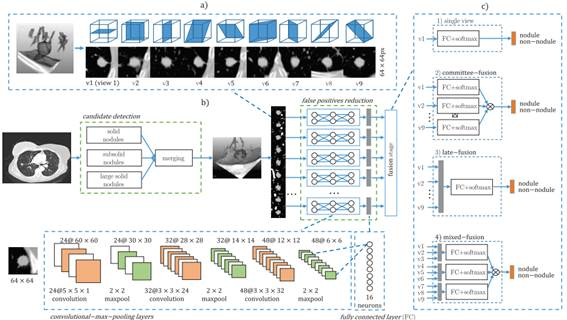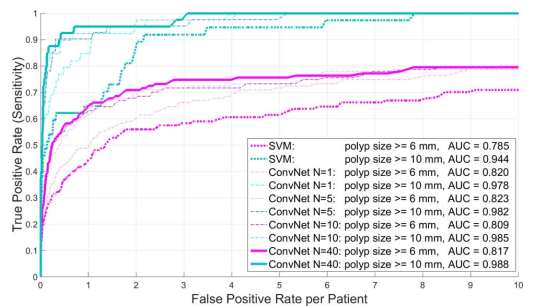### 2、图像分割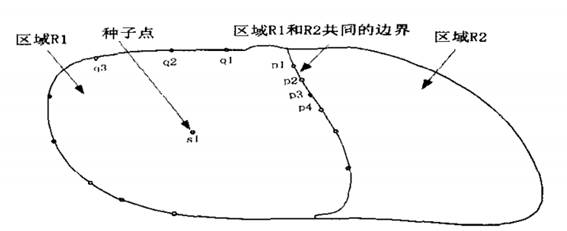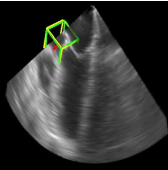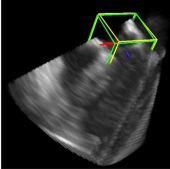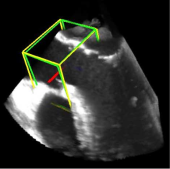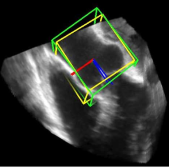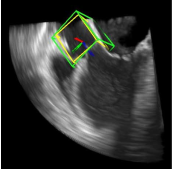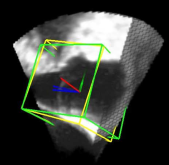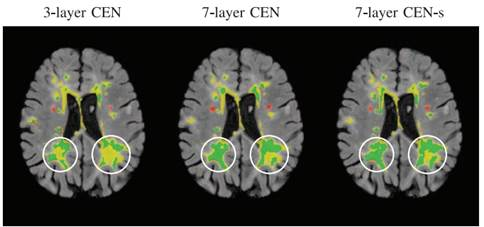2018年德国医疗康复机构提出一种具有代表性的基于全卷积的前列腺图像分割方法。用CNN在前列腺的MRI图像上进行端到端训练，并可以一次完成整个分割。提出了一种新的目标函数，在训练期间根据Dice系数进行优化。通过这种方式，可以处理前景和背景之间存在不平衡的情况，并且增加了随机应用的数据非线性变换和直方图匹配。实验评估中表明，该方法在公开数据集上取得了优秀的结果，但大大降低了处理时间。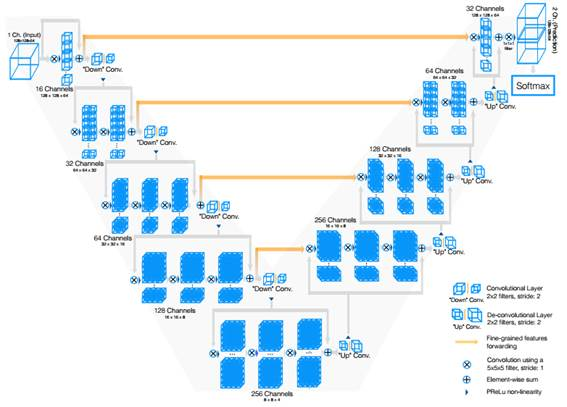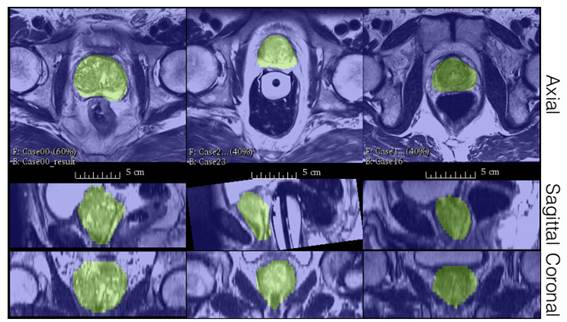### 3、图像配准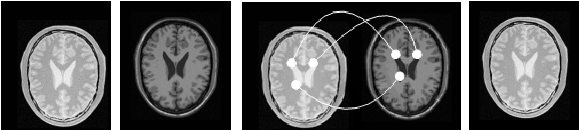​ （a） （b） （c） （d）

2019年华中科技大学对基于PCANet的结构非刚性多模医学图像配准展开研究。提出了一种基于PCANet的结构表示方法用于多模态医学图像配准。与人工设计的特征提取方法相比，PCANet可以通过多级线性和非线性变换自动从大量医学图像中学习内在特征。所提出的方法可以通过利用PCANet的各个层中提取的多级图像特征来为多模态图像提供有效的结构表示。对Atlas，BrainWeb和RIRE数据集的大量实验表明，与MIND，ESSD，WLD和NMI方法相比，所提出的方法可以提供更低的TRE值和更令人满意的结果。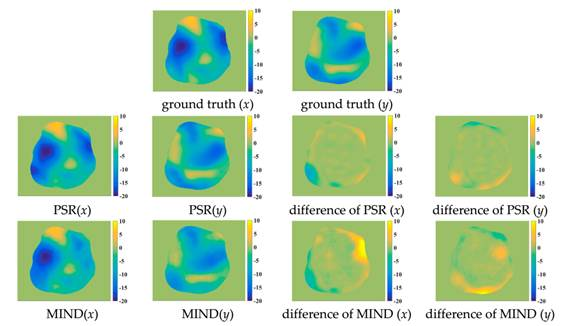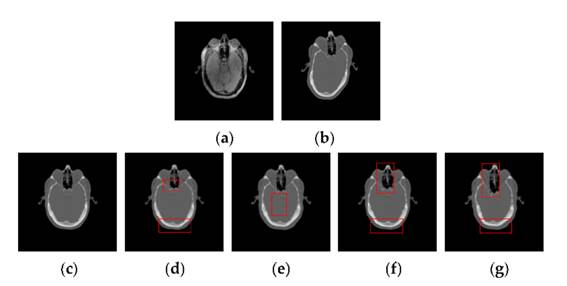### 4、图像融合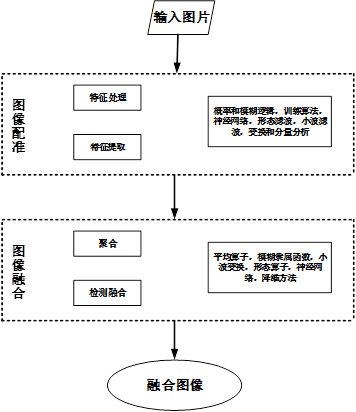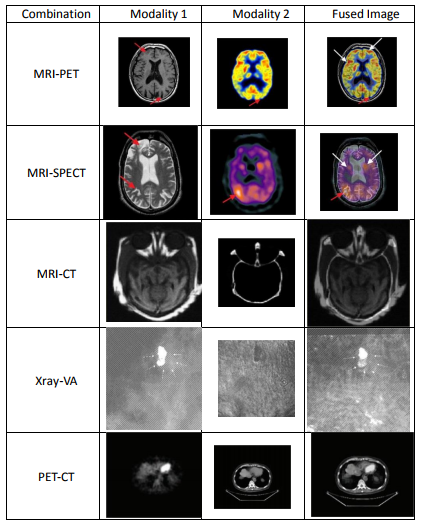### 5、预测与挑战

1）数据维度问题-2D与3D：在迄今为止的大多数工作中，是在2D图像中进行处理分析。人们常常质疑向3D过渡是否是迈向性能提高的重要一步。数据增强过程中存在若干变体，包括2.5D。例如，在Roth等人的研究中，以结肠息肉或淋巴结候选体中的体素为中心截取轴向图像，存在冠状和矢状图像。

2）学习方法 - 无监督与监督：当我们查看网络文献时，很明显大多数工作都集中在受监督的CNN上，以实现分类。这种网络对于许多应用是重要的，包括检测，分割和标记。尽管如此，一些工作仍集中于无监督方案，这些方案主要表现为图像编码。诸如玻尔兹曼机器（RBM）之类的无监督表示学习方法可能胜过滤波器，因为它们直接从训练数据中学习特征描述。RBM通过生成学习目标进行培训；这使网络成为可能从未标记的数据中学习，但不一定产生最适合分类的特征。Van Tulder等人进行了一项调查，结合卷积分类和RBM的生成和判别学习目标的优点，该机器学习了对描述训练数据和分类都很好的过滤器。结果表明，学习目标的组合完全胜过生成性学习。

3）迁移学习和微调：在医学成像领域中获取与ImageNet一样全面注释的数据集仍然是一个挑战。当没有足够的数据时，有几种方法可以继续：1）迁移学习：从自然图像数据集或不同医学领域预训练的CNN模型（监督）用于新的医疗任务。在一个方案中，预先训练CNN应用于输入图像，然后从网络层提取输出。提取的输出被认为是特征并且用于训练单独的模式分类器。2）微调：当手头的任务确实存在中等大小的数据集时，较好的方案是使用预先训练的CNN作为网络的初始化，然后进行进一步的监督训练，其中几个（或全部）网络层，使用任务的新数据。

4）数据隐私受社会和技术问题的影响，需要从社会学和技术学的角度共同解决。在卫生部门讨论隐私时，会想到HIPAA（1996年健康保险流通与责任法案）。它为患者提供有关保护个人身份信息的法律权利，并为医疗保健提供者承担保护和限制其使用或披露的义务。在医疗保健数据不断增加的同时，研究人员面临如何加密患者信息以防止其被使用或披露的问题。同时带来，限制访问数据可能遗漏非常重要的信息。

### 参考文献

林晓, 邱晓嘉. 图像分析技术在医学上的应用 [J] . 包头医学院学报, 2005, 21 (3) ： 311~ 314

周贤善. 医学图像处理技术综述[J]. 福建电脑, 2009(1):34-34.

Mcinerney T , Terzopoulos D . Deformable models in medical image analysis: a survey[J]. Medical Image Analysis, 1996, 1(2):91.

Litjens G , Kooi T , Bejnordi B E , et al. A survey on deep learning in medical image analysis[J]. Medical Image Analysis, 2017, 42:60-88.

Deserno T M , Heinz H , Maier-Hein K H , et al. Viewpoints on Medical Image Processing: From Science to Application[J]. Current Medical Imaging Reviews, 2013, 9(2):79-88.

A. Setio et al., “Pulmonary nodule detection in CT images using multiview convolutional networks,” IEEE Trans. Med. Imag., vol. 35, no. 5,pp. 1160–1169, May 2016.

H. Roth et al., “Improving computer-aided detection using convolutional neural networks and random view aggregation,” IEEE Trans.Med. Imag., vol. 35, no. 5, pp. 1170–1181, May 2016

林瑶, 田捷. 医学图像分割方法综述[J]. 模式识别与人工智能, 2002, 15(2).

Ghesu F C , Georgescu B , Mansi T , et al. An Artificial Agent for Anatomical Landmark Detection in Medical Images[C]// International Conference on Medical Image Computing & Computer-assisted Intervention. Springer, Cham, 2016.

Pham D L , Xu C , Prince J L . Current methods in medical image segmentation.[J]. Annual Review of Biomedical Engineering, 2000, 2(2):315-337.

Lehmann T M , Gonner C , Spitzer K . Survey: interpolation methods in medical image processing[J]. IEEE Transactions on Medical Imaging, 1999, 18(11):1049-1075.

Cootes T F , Taylor C J . Statistical Models of Appearance for Medical Image Analysis and Computer Vision[J]. Proceedings of SPIE - The International Society for Optical Engineering, 2001, 4322(1).

 T. Brosch et al., “Deep 3D convolutional encoder networks with shortcuts for multiscale feature integration applied to multiple sclerosis lesion segmentation,” IEEE Trans. Med. Imag., vol. 35, no. 5,pp. 1229–1239, May 2016.

Ghesu F C , Krubasik E , Georgescu B , et al. Marginal Space Deep Learning: Efficient Architecture for Volumetric Image Parsing[J]. IEEE Transactions on Medical Imaging, 2016, 35(5):1217-1228.

Milletari F , Navab N , Ahmadi S A . V-Net: Fully Convolutional Neural Networks for Volumetric Medical Image Segmentation[J]. 2016.

 .周永新, 罗述谦. 一种人机交互式快速脑图象配准系统[J] . 北京生物医学工程, 2002; 21 (1) ：11~14

杨虎, 马斌荣, 任海萍. 基于互信息的人脑图象配准研究[J] . 中国医学物理学杂志, 2001; 18 (2) ：69~73

汪家旺，愈同福，姜晓彤，等.肺部孤立性结节定量研究[J].中国医学影 像技术,2003,19(9)：1218~1219

Ishihara S , Ishihara K , Nagamachi M , et al. An analysis of Kansei structure on shoes using self-organizing neural networks[J]. International Journal of Industrial Ergonomics, 1997, 19(2):93-104.

Maintz J B , Viergever M A . A Survey of Medical Image Registration[J]. Computer & Digital Engineering, 2009, 33(1):140-144.

Hill D L G , Batchelor P G , Holden M , et al. Medical image registration[J]. Physics in Medicine & Biology, 2008, 31(4):1-45.

Razzak M I , Naz S , Zaib A . Deep Learning for Medical Image Processing: Overview, Challenges and Future[J]. 2017.

林晓, 邱晓嘉. 图像分析技术在医学上的应用 [J] . 包头医学院学报, 2005, 21 (3) ： 311~ 314

## MTTM：在深度图像上基于多任务模板匹配方法的目标检测，分割和姿势估计

2020-01-14 19:59:20 john_bh 阅读数 525
• ###### matlab 基于阈值分割的区域生长的医学图像分割3讲

matlab 基于阈值分割的区域生长的医学图像分割3讲 </p>

3课时 23分钟 614人学习 刘昱显
免费试看

Pose Estimation Using Depth Images

code:

## 1.Introduction

• 一种新颖的基于深度的框架MTTM，它与NN模板匹配，并使用共享特征图预测对象的分割蒙版和姿态，而无需对新对象进行额外的训练。
• 无需对象与场景的任何对齐即可生成分割蒙版，这通过排除不属于对象一部分的点而增强了姿态估计性能的鲁棒性。
• 实验结果表明MTTM优于使用颜色的基线方法。## 3. Method

### B.Network Architecture### C.Learning Networks for Multiple Tasks## 4. Experiments

### B.Evaluation of Segmentation### C.Evaluation of Object Detection and Pose Estimation### D.Real Templates and New Objects## [SemanticSoftSegmentation：语义软分割]-2018最强图像分割光谱消光算法调研与测试

2018-11-27 19:07:06 lovebyz 阅读数 2629
• ###### matlab 基于阈值分割的区域生长的医学图像分割3讲

matlab 基于阈值分割的区域生长的医学图像分割3讲 </p>

3课时 23分钟 614人学习 刘昱显
免费试看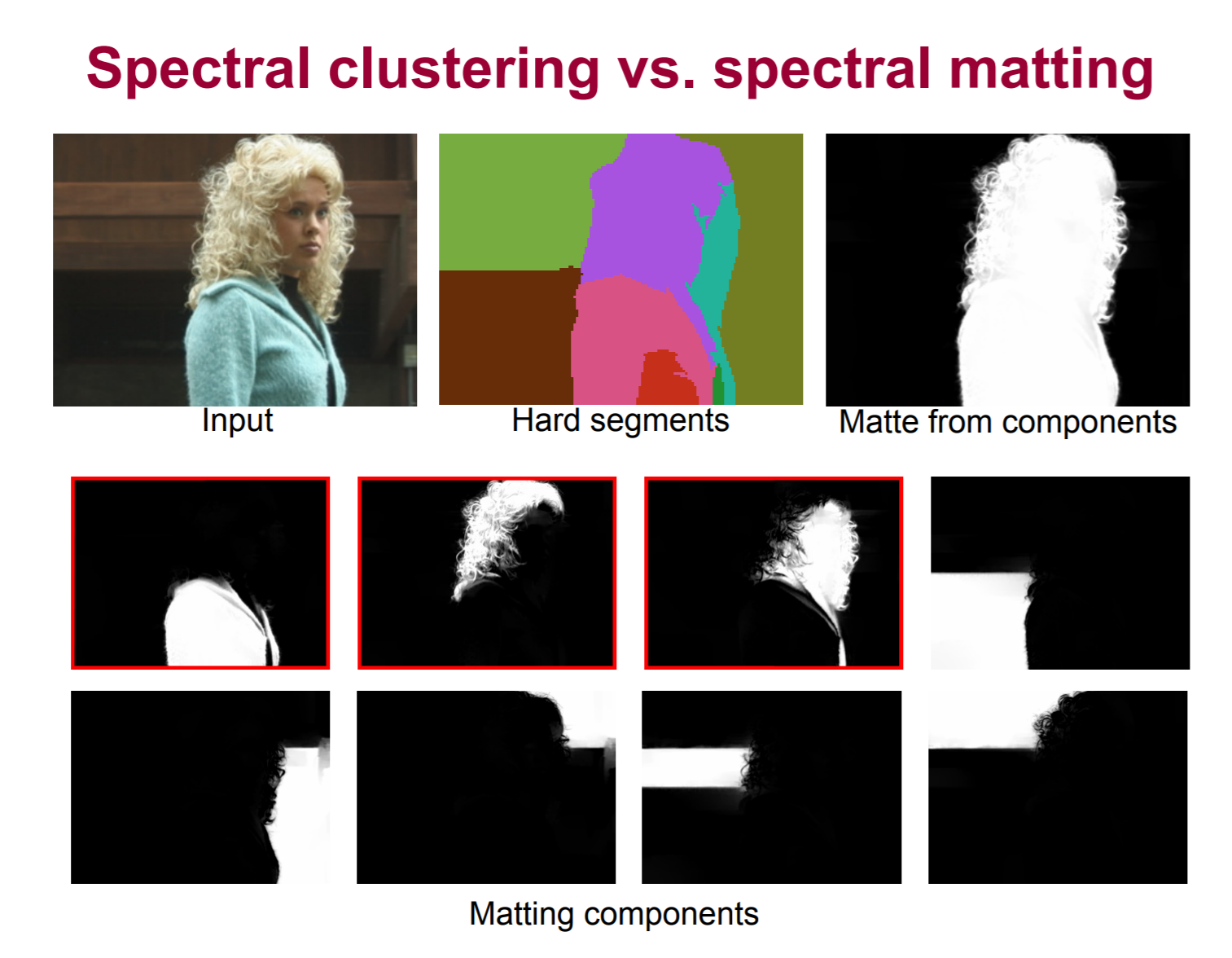Github项目：https://github.com/yaksoy/SemanticSoftSegmentation

• 特征降维，从128维特征降到3维。
• 超像素处理，用于计算Superpixels。
• 计算仿射变换和 Laplacian，包括：Matting Affinity，semantic affinity 和 non-local
color affinity 以及 affinityMatrixToLaplacian.
• 计算 Laplacian 的特征分解，得到 100 个特征向量(eigenvectors).
• 初始化优化，主要计算初始化 soft segments，对特征进行语义初始化；关于深度网络提取的特征进行 Group segments.
• 最终优化Final optimization，sparsification.

## 简介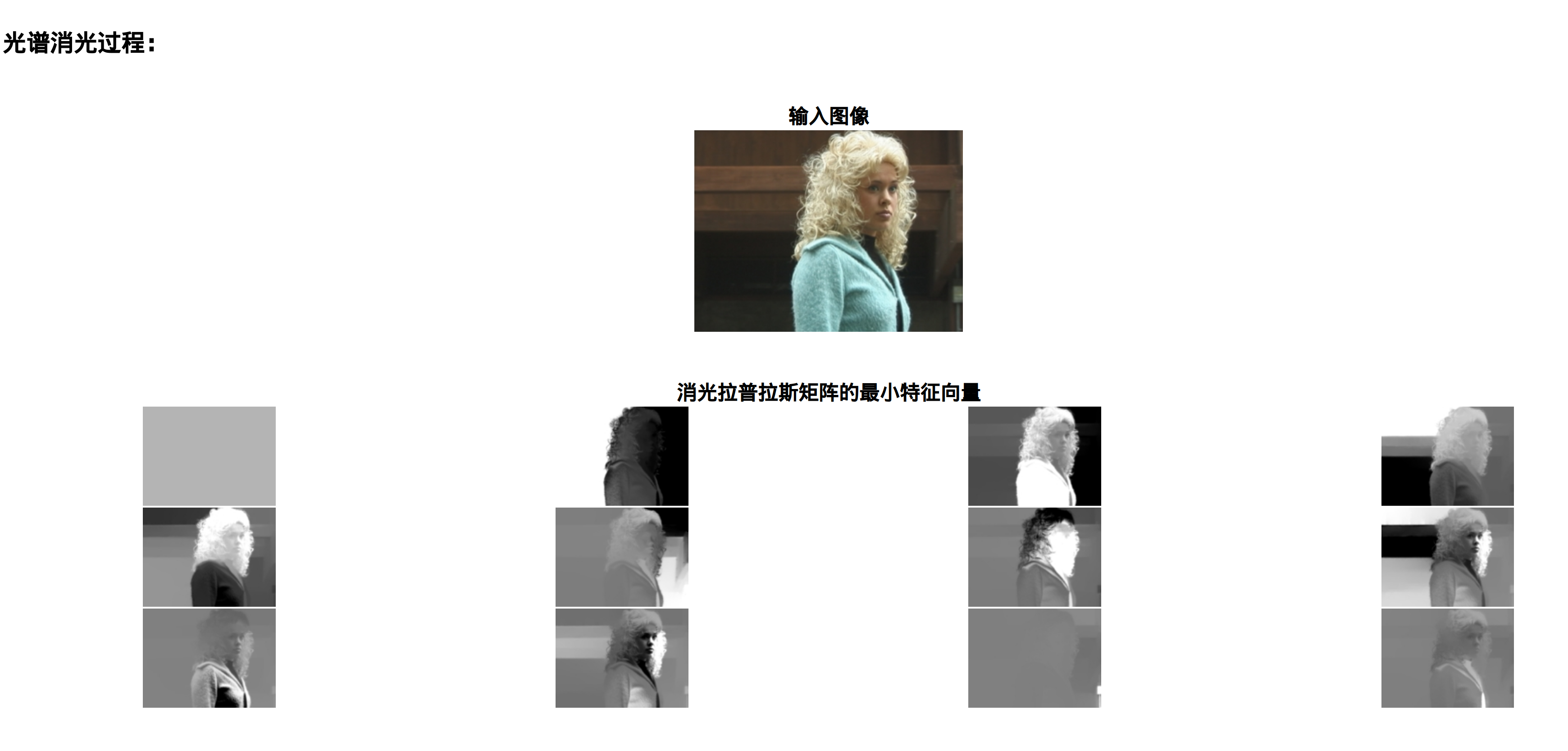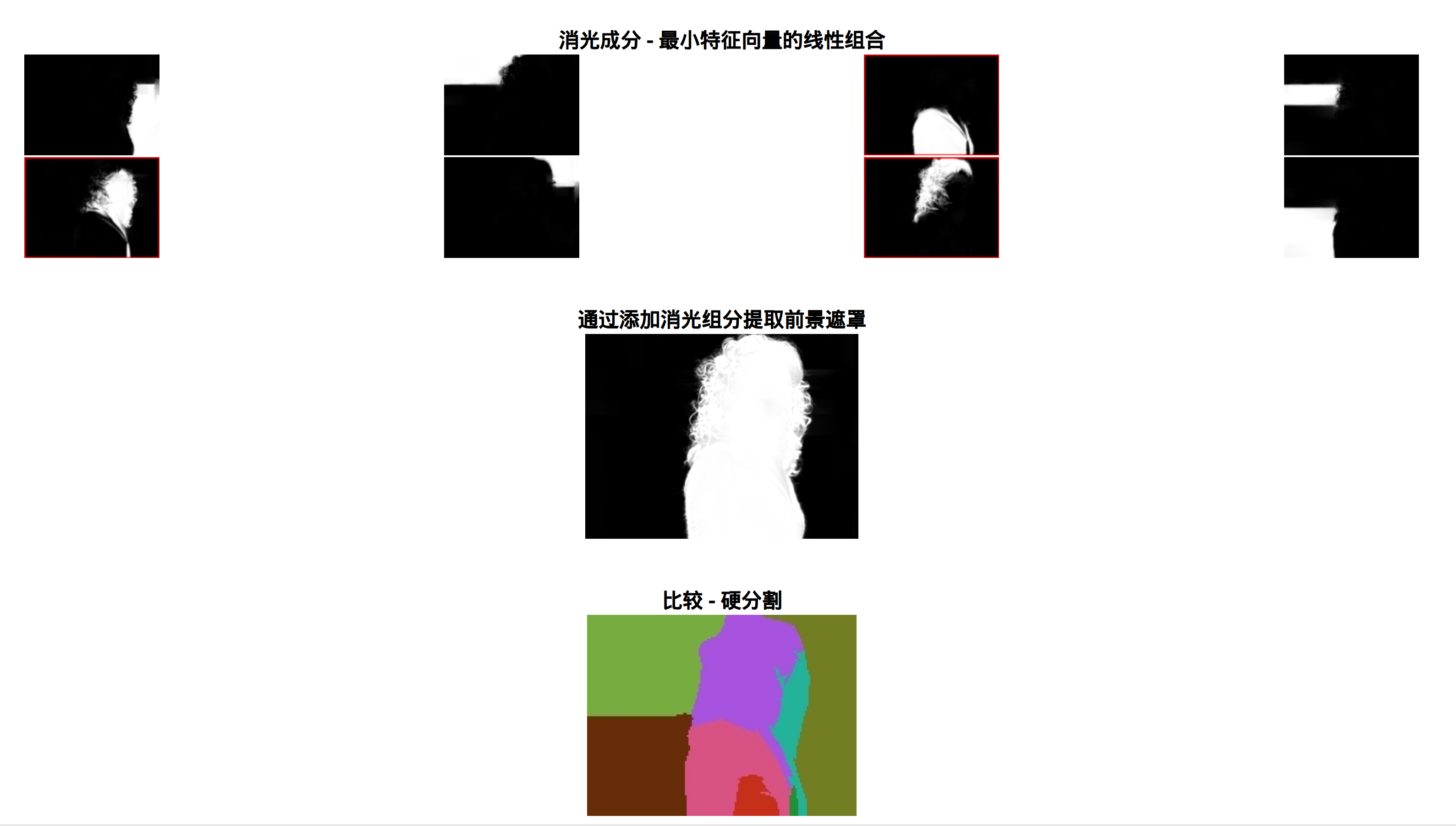## 方法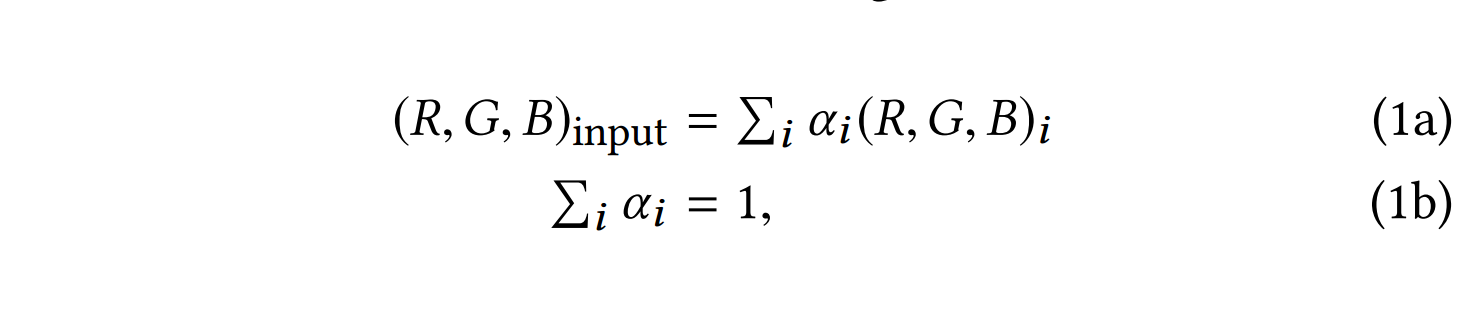## 背景

### 光谱消光算法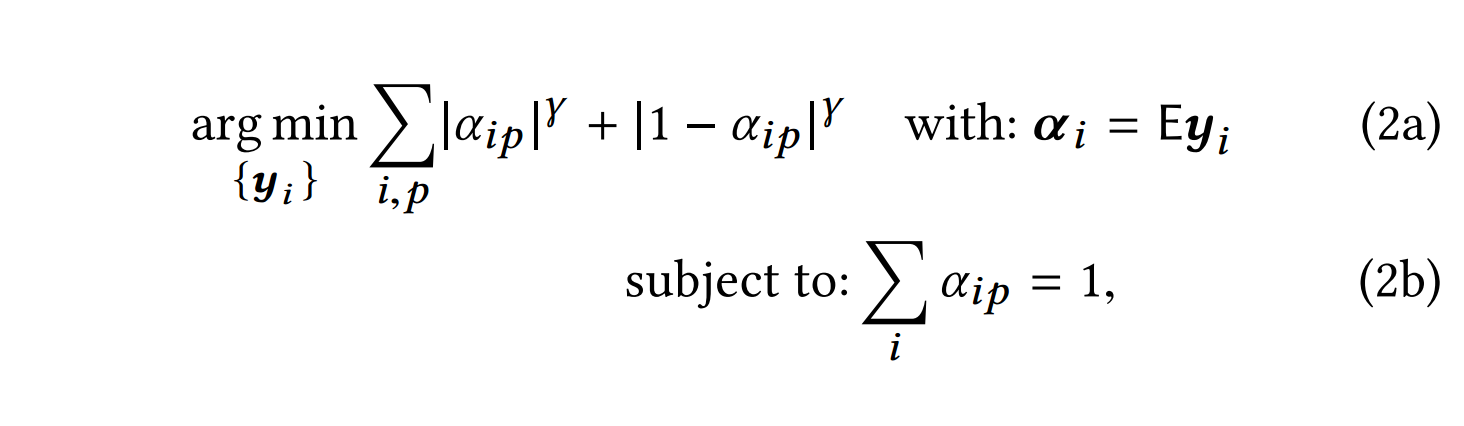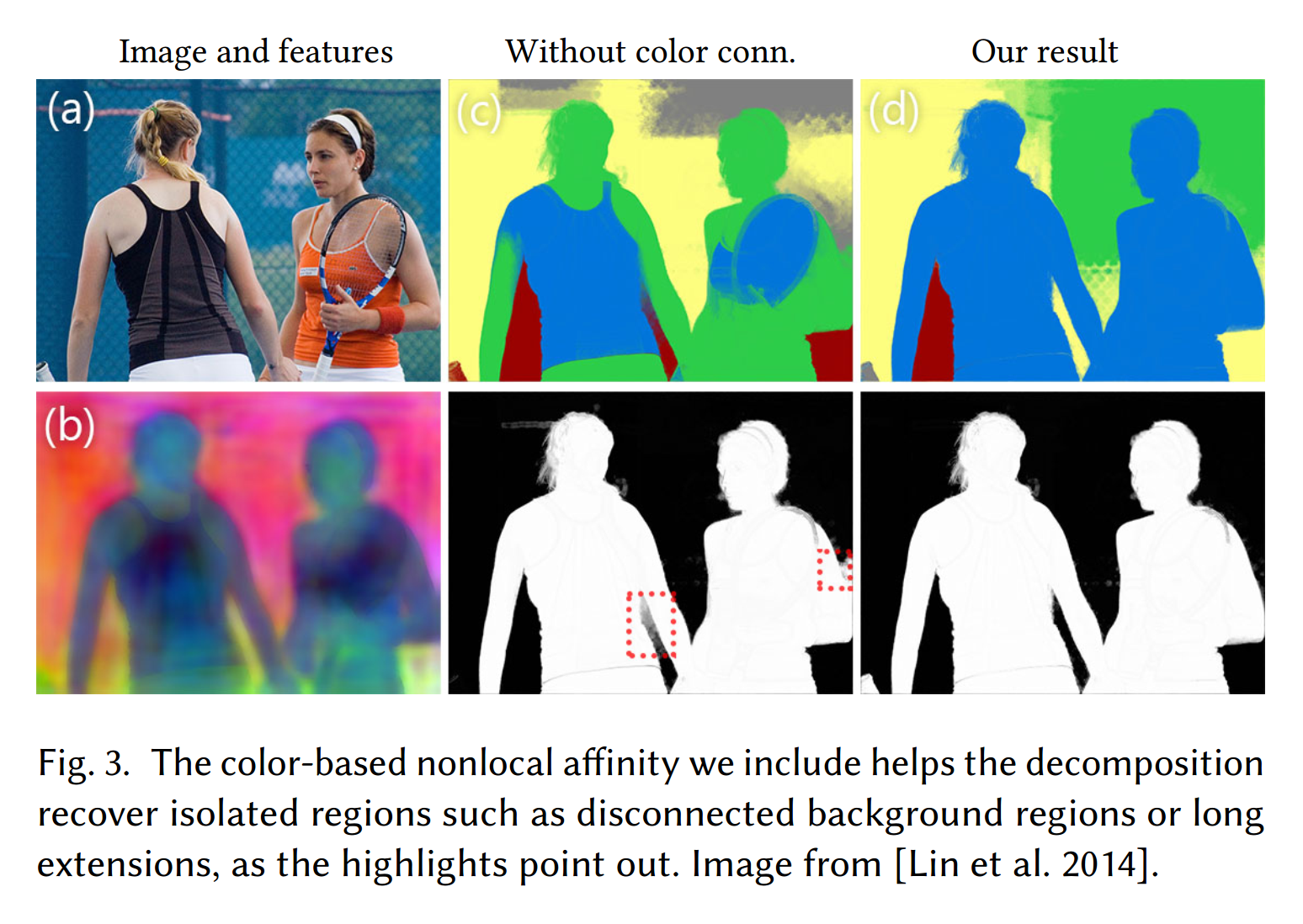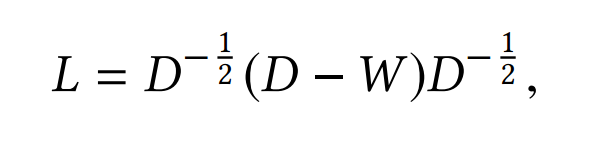### 非局部颜色亲和力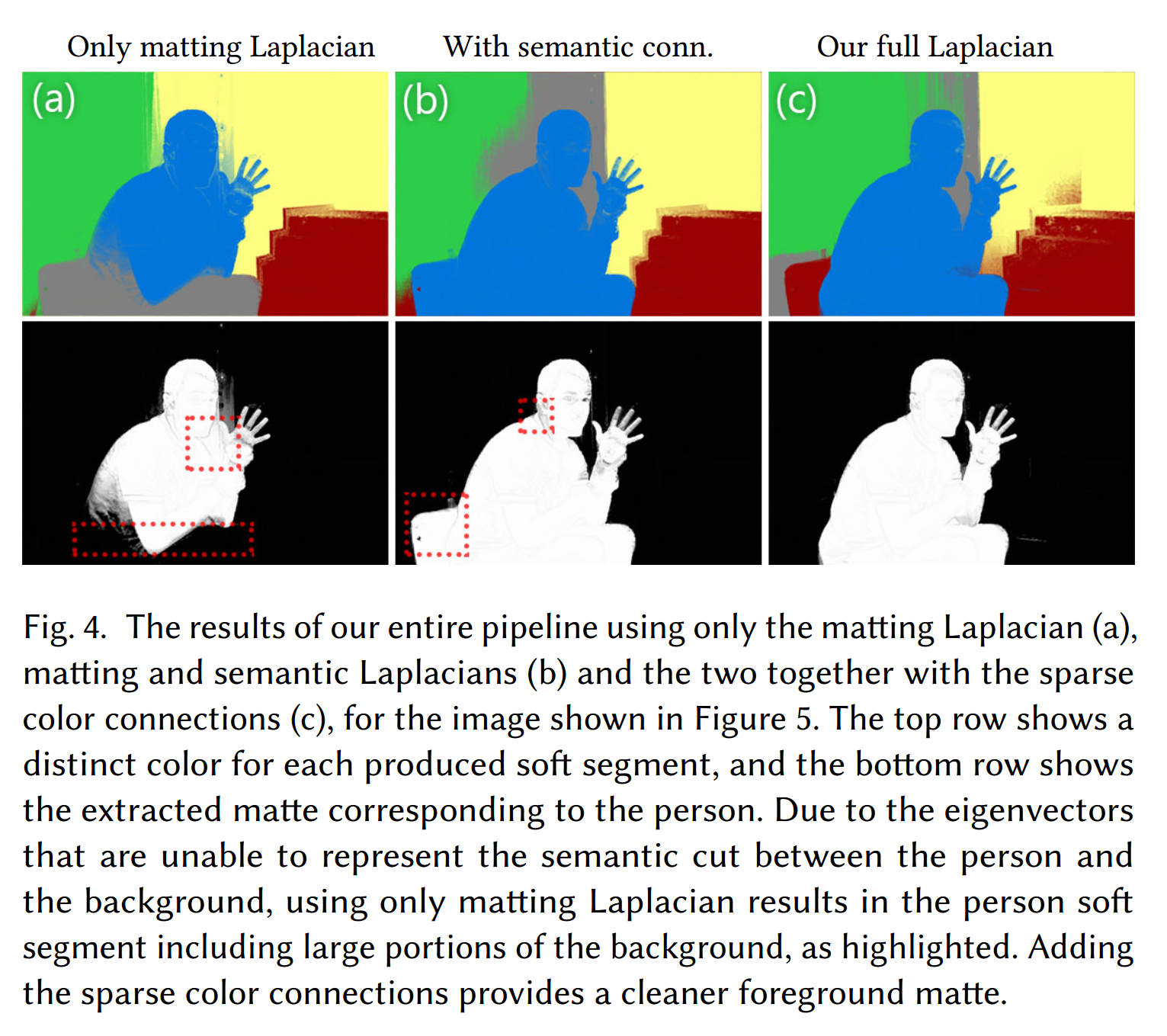。。。

### 创建图层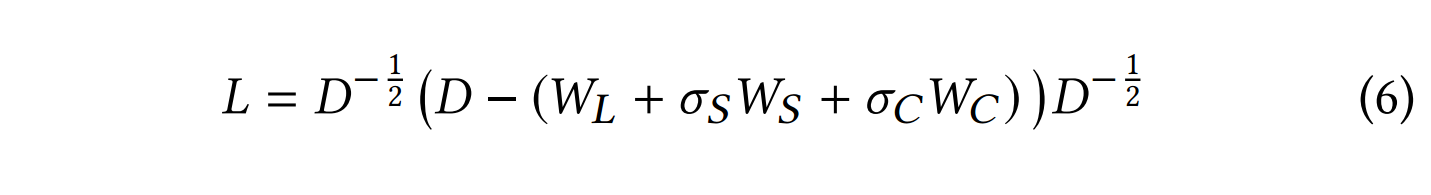## 实验分析

### 光谱消光和语义分割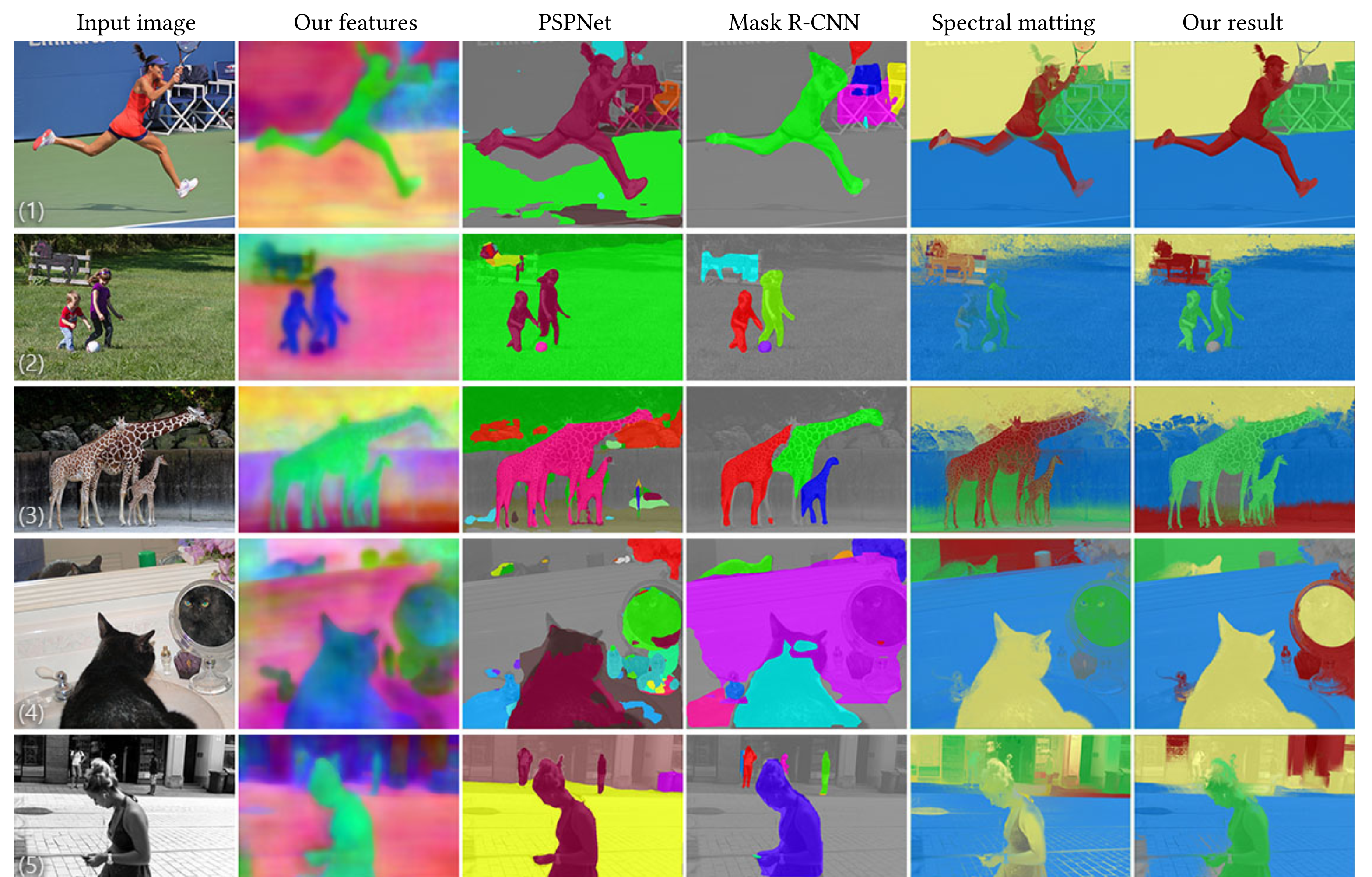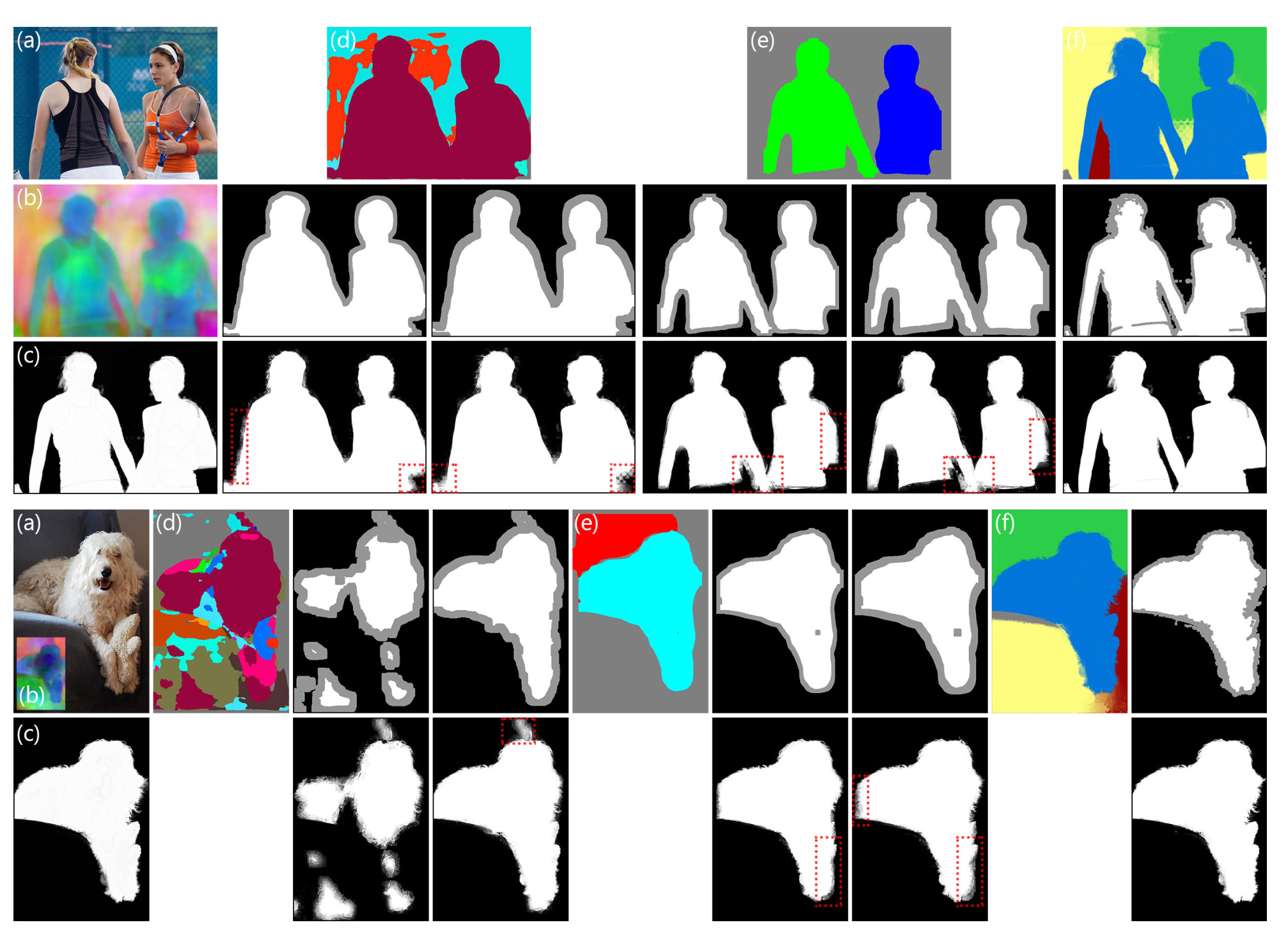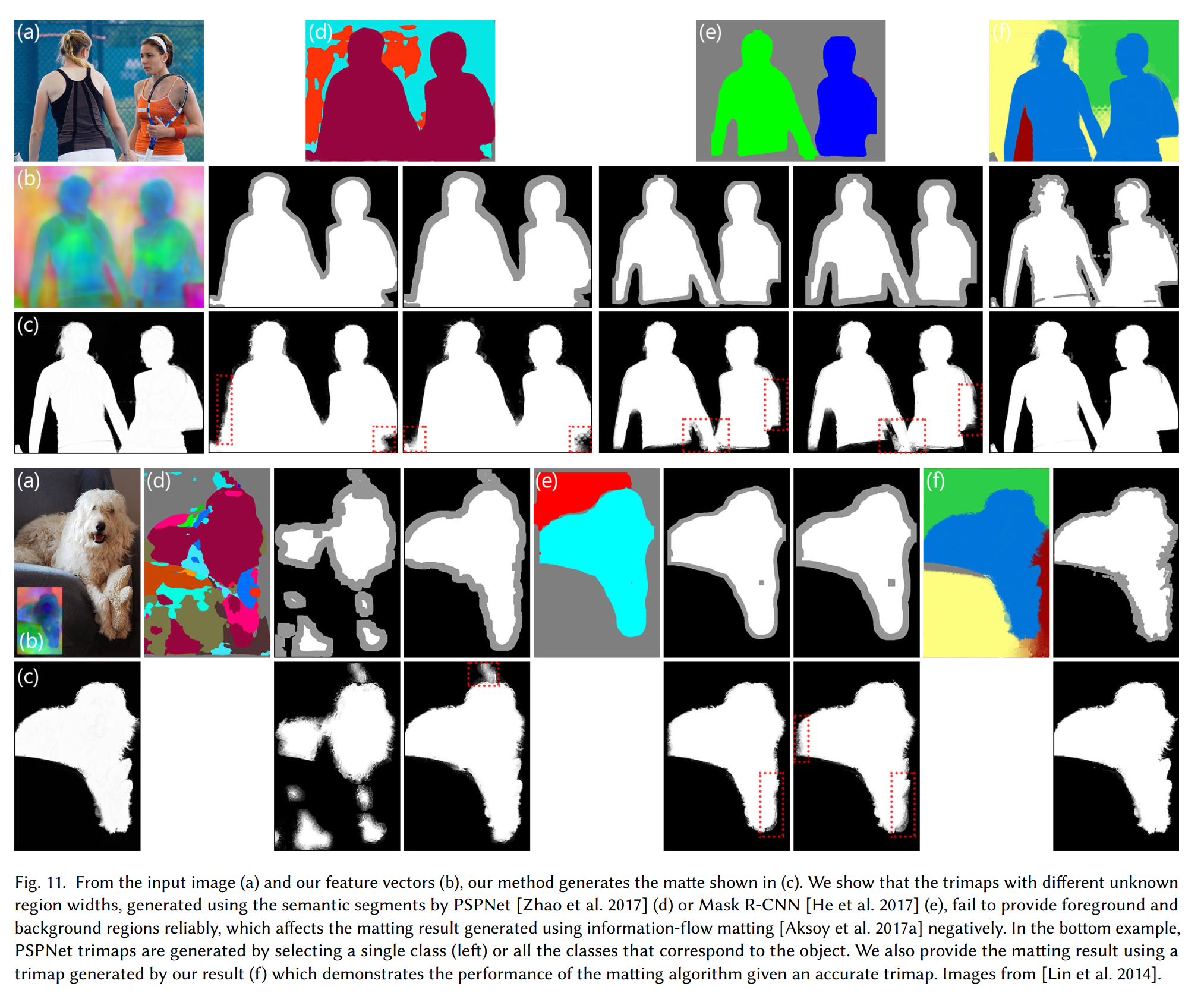### 自然图像消光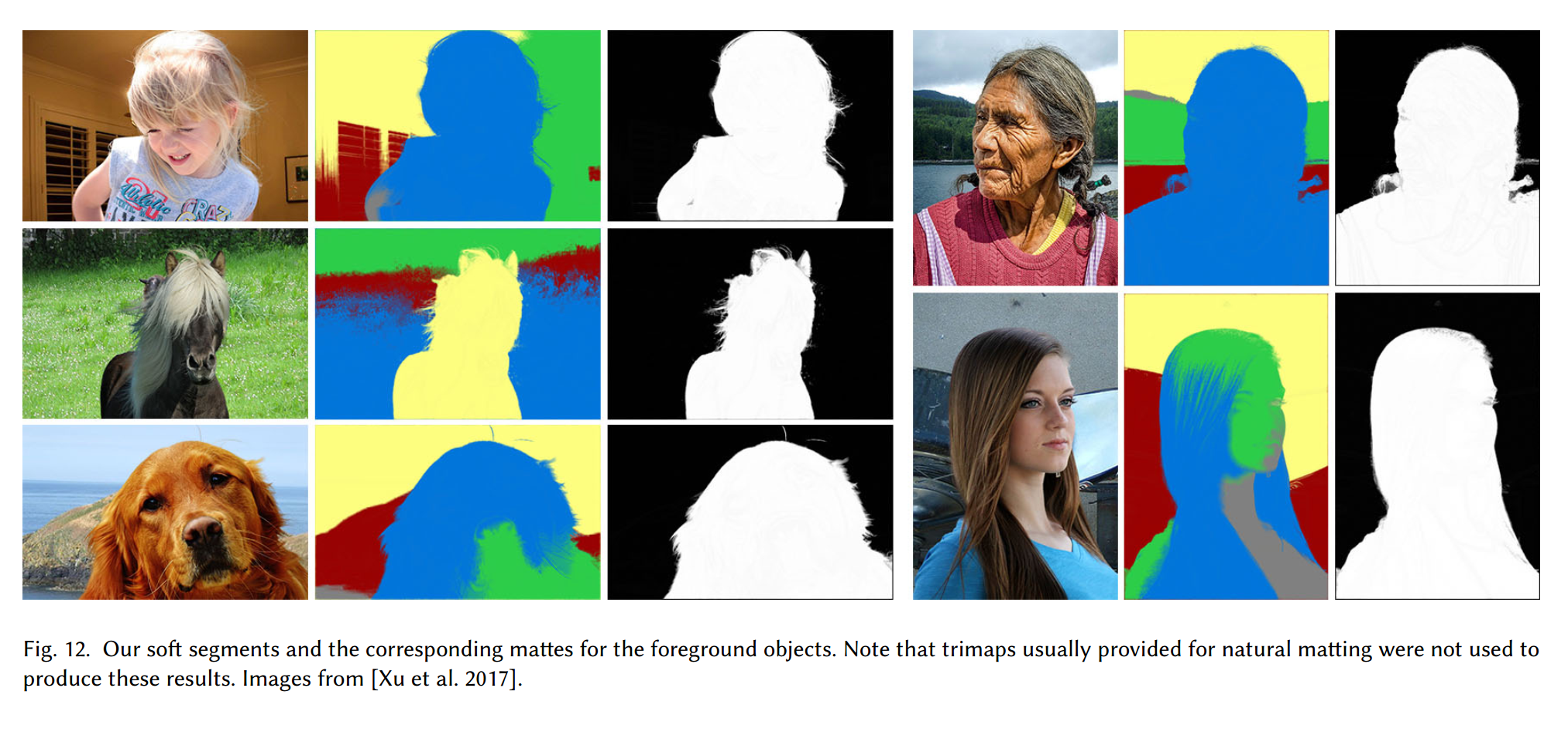### 软色分割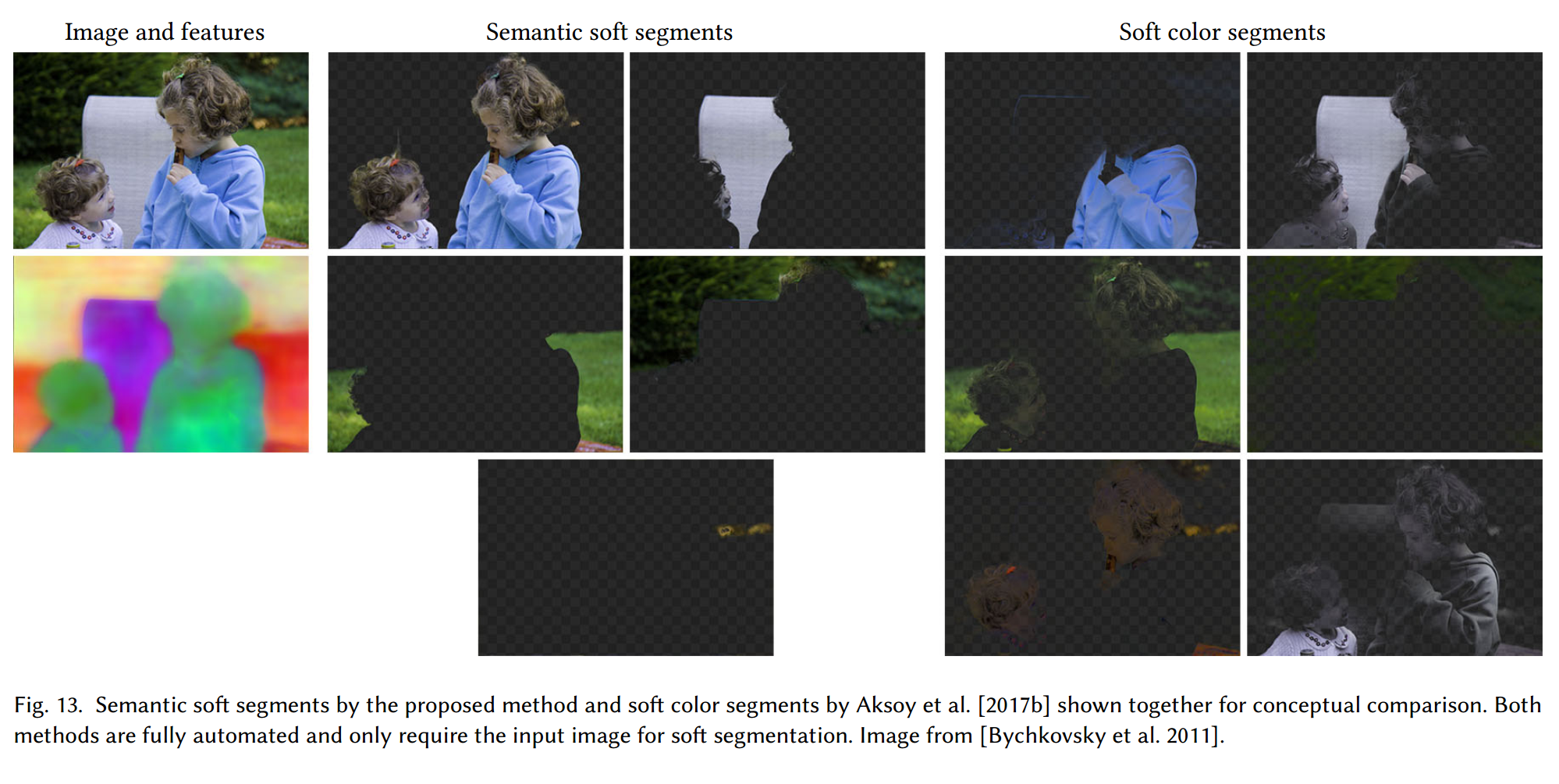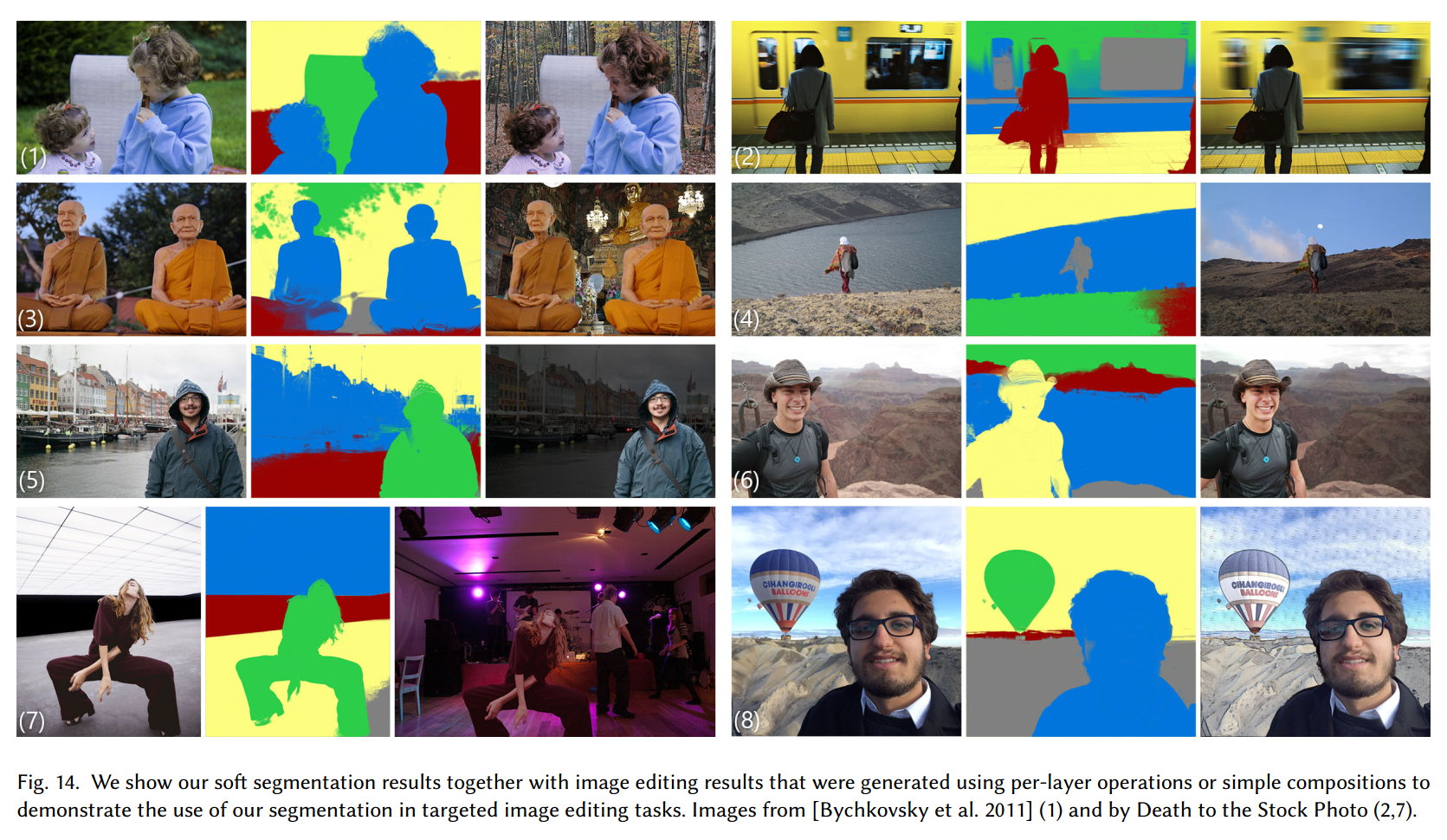## 限制和未来的工作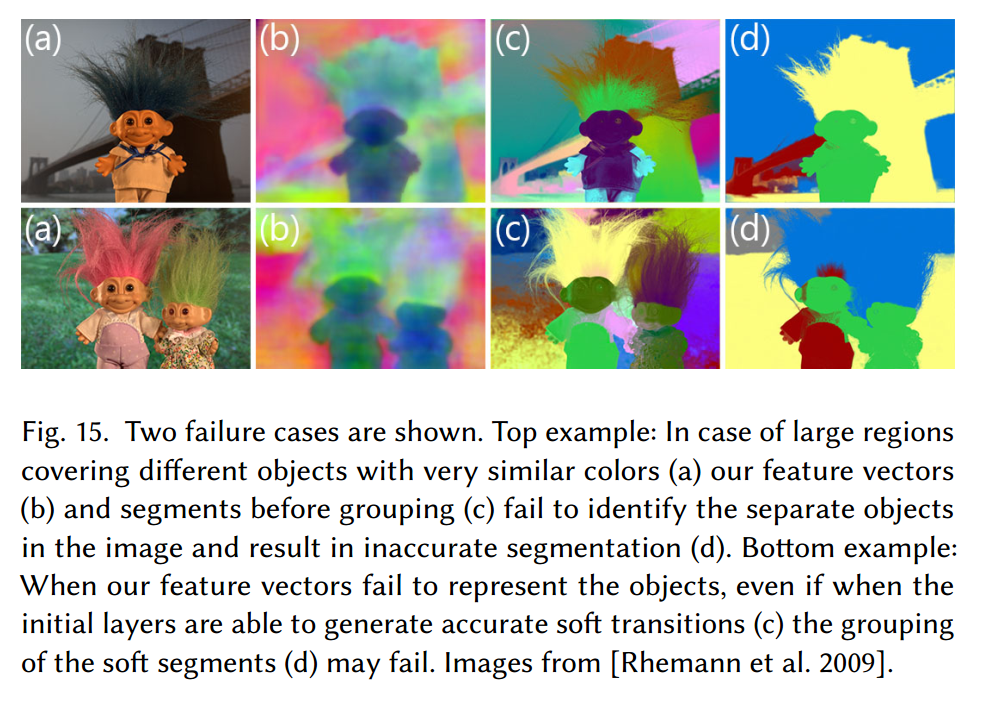## 结论

Semantic Soft Segmentation
Computing affinities
Computing eigenvectors
Initial optimization
Computing k-means initialization using semantic features...
Starting optimization...
Iteration 10 of 40
Iteration 20 of 40
Iteration 30 of 40
Iteration 40 of 40
Final optimization
Iteration 5 of 20
Iteration 10 of 20
Iteration 15 of 20
Iteration 20 of 20
pcg 在解的 迭代 17 处收敛，并且相对残差为 6.9e-07。
Done.
Spectral Matting
Computing affinities
Computing eigenvectors
Optimization
Computing k-means initialization...
Starting optimization...
Iteration 10 of 20
Iteration 20 of 20
Done.
>>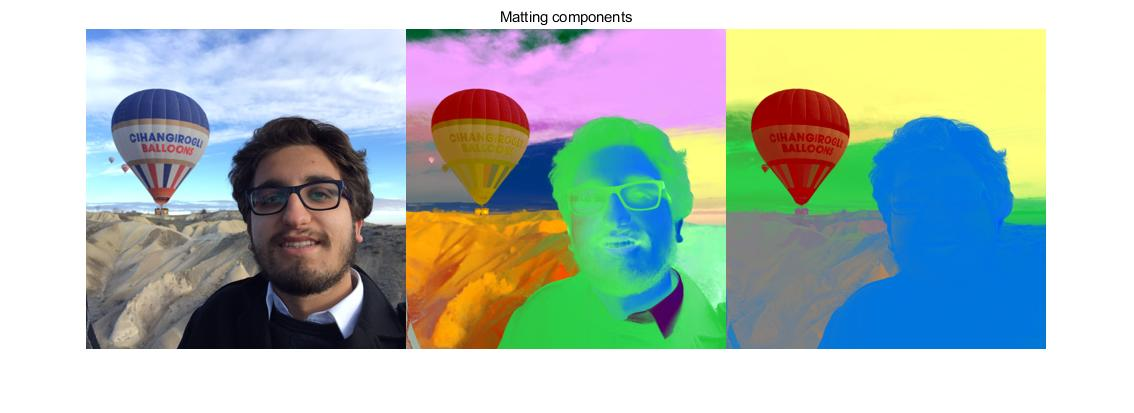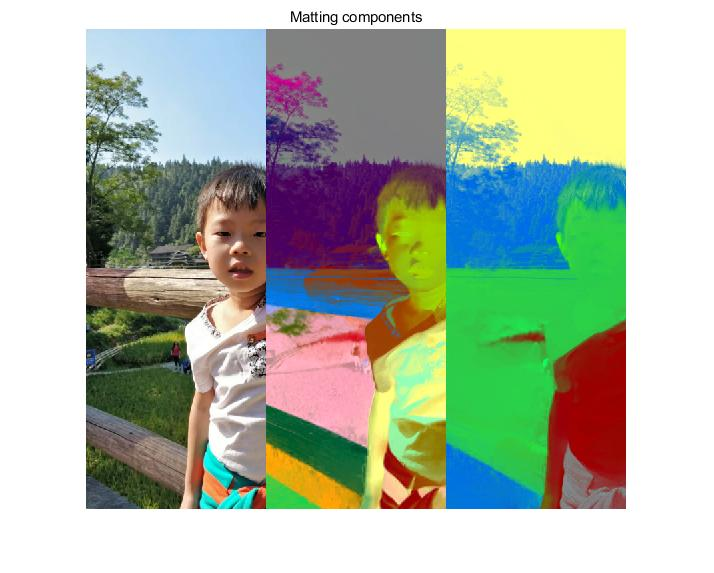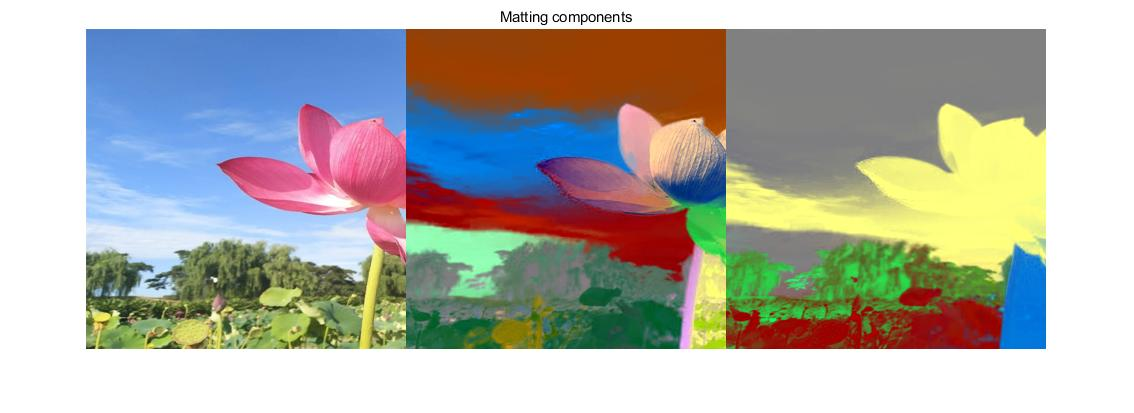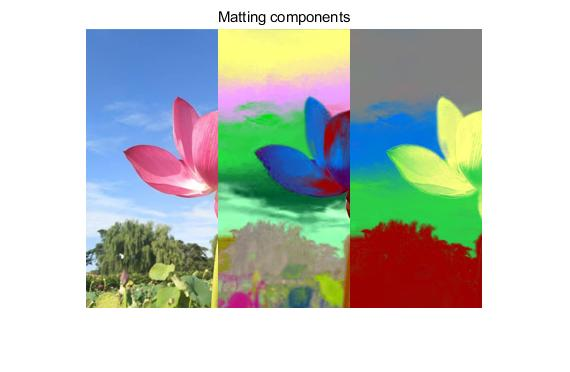## OpenCV里常用的图像处理

2017-01-05 09:33:43 DaveBobo 阅读数 3258
• ###### matlab 基于阈值分割的区域生长的医学图像分割3讲

matlab 基于阈值分割的区域生长的医学图像分割3讲 </p>

3课时 23分钟 614人学习 刘昱显
免费试看

# cv篇包含以下几个部分：

1、图像处理

Sobel

void cvSobel( const CvArr* src, CvArr* dst, int xorder, int yorder, int aperture_>

src

dst

xorder

x 方向上的差分阶数

yorder

y 方向上的差分阶数

aperture_size

Laplace ：计算图像的 Laplacian 变换

void cvLaplace( const CvArr* src, CvArr* dst, int aperture_>

src : 输入图像.

dst : 输出图像.

aperture_size : 核大小 (与 cvSobel 中定义一样).

Canny：采用 Canny算法做边缘检测

void cvCanny( const CvArr* image, CvArr* edges, double threshold1,

double threshold2, int aperture_>

image : 单通道输入图像.

edges : 单通道存储边缘的输出图像

threshold1 : 第一个阈值

threshold2 : 第二个阈值

aperture_size : Sobel 算子内核大小 (见 cvSobel).

• 注意事项：cvCanny只接受单通道图像作为输入。

PreCornerDetect：计算用于角点检测的特征图，

void cvPreCornerDetect( const CvArr* image, CvArr* corners, int aperture_>

image : 输入图像.

corners : 保存候选角点的特征图

aperture_size : Sobel 算子的核大小(见cvSobel).

// 假设图像格式为浮点数

IplImage* corners = cvCloneImage(image);

IplImage* dilated_corners = cvCloneImage(image);

IplImage* corner_mask = cvCreateImage( cvGetSize(image), 8, 1 );

cvPreCornerDetect( image, corners, 3 );

cvDilate( corners, dilated_corners, 0, 1 );

cvSub( corners, dilated_corners, corners );

cvCmpS( corners, 0, corner_mask, CV_CMP_GE );

cvReleaseImage( &corners );

cvReleaseImage( &dilated_corners );

CornerEigenValsAndVecs：计算图像块的特征值和特征向量，用于角点检测

void cvCornerEigenValsAndVecs( const CvArr* image, CvArr* eigenvv,

int block_size, int aperture_>

image : 输入图像.

eigenvv : 保存结果的数组。必须比输入图像宽 6 倍。

block_size : 邻域大小 (见讨论).

aperture_size : Sobel 算子的核尺寸(见 cvSobel).

λ1, λ2 - M 的特征值，没有排序

(x1, y1) - 特征向量，对 λ1

(x2, y2) - 特征向量，对 λ2

CornerMinEigenVal：计算梯度矩阵的最小特征值，用于角点检测

void cvCornerMinEigenVal( const CvArr* image, CvArr* eigenval, int block_size, int aperture_>

image : 输入图像.

eigenval : 保存最小特征值的图像. 与输入图像大小一致

block_size : 邻域大小 (见讨论 cvCornerEigenValsAndVecs).

aperture_size : Sobel 算子的核尺寸(见 cvSobel). 当输入图像是浮点数格式时，该参数表示用来计算差分固定的浮点滤波器的个数.

CornerHarris

image : 输入图像。

harris_responce : 存储哈里斯（Harris）检测responces的图像。与输入图像等大。

block_size : 邻域大小（见关于cvCornerEigenValsAndVecs的讨论）。

aperture_size : 扩展 Sobel 核的大小（见 cvSobel）。格式. 当输入图像是浮点数格式时，该参数表示用来计算差分固定的浮点滤波器的个数。

k----harris 检测器的自由参数。参见下面的公式。

det(M) - k*trace(M)2 （这里2是平方）

FindCornerSubPix:精确角点位置

void cvFindCornerSubPix( const CvArr* image, CvPoint2D32f* corners,

int count, CvSize win, CvSize zero_zone,

CvTermCriteria criteria );

image : 输入图像.

corners : 输入角点的初始坐标，也存储精确的输出坐标

count : 角点数目

win : 搜索窗口的一半尺寸。如果 win=(5,5) 那么使用 5*2+1 × 5*2+1 = 11 × 11 大小的搜索窗口

zero_zone : 死区的一半尺寸，死区为不对搜索区的中央位置做求和运算的区域。它是用来避免自相关矩阵出现的某些可能的奇异性。当值为 (-1,-1) 表示没有死区。

criteria:求角点的迭代过程的终止条件。即角点位置的确定，要么迭代数大于某个设定值，或者是精确度达到某个设定值。 criteria 可以是最大迭代数目，或者是设定的精确度，也可以是它们的组合。

εi=DIpiT•(q-pi)

sumi(DIpi•DIpiT)•q - sumi(DIpi•DIpiT•pi) = 0

q=G-1•b

GoodFeaturesToTrack:确定图像的强角点

void cvGoodFeaturesToTrack( const CvArr* image, CvArr* eig_image, CvArr* temp_image,

CvPoint2D32f* corners, int* corner_count,

double quality_level, double min_distance,

image : 输入图像，8-位或浮点32-比特，单通道

eig_image : 临时浮点32-位图像，尺寸与输入图像一致

temp_image : 另外一个临时图像，格式与尺寸与 eig_image 一致

corners : 输出参数，检测到的角点

corner_count : 输出参数，检测到的角点数目

quality_level : 最大最小特征值的乘法因子。定义可接受图像角点的最小质量因子。

min_distance : 限制因子。得到的角点的最小距离。使用 Euclidian 距离

InitLineIterator : 初始化线段迭代器

int cvInitLineIterator( const CvArr* image, CvPoint pt1, CvPoint pt2,

CvLineIterator* line_iterator, int connectivity=8 );

image : 带采线段的输入图像.

pt1 : 线段起始点

pt2 : 线段结束点

line_iterator : 指向线段迭代器状态结构的指针

connectivity : 被扫描线段的连通数，4 或 8.

CvScalar sum_line_pixels( IplImage* image, CvPoint pt1, CvPoint pt2 )

{

CvLineIterator iterator;

int blue_sum = 0, green_sum = 0, red_sum = 0;

int count = cvInitLineIterator( image, pt1, pt2, &iterator, 8 );

for( int i = 0; i < count; i++ ){

blue_sum += iterator.ptr;

green_sum += iterator.ptr;

red_sum += iterator.ptr;

CV_NEXT_LINE_POINT(iterator);

/* print the pixel coordinates: demonstrates how to calculate the coordinates */

{

int offset, x, y;

/* assume that ROI is not set, otherwise need to take it into account. */

offset = iterator.ptr - (uchar*)(image->imageData);

y = offset/image->widthStep;

x = (offset - y*image->widthStep)/(3*sizeof(uchar) /* size of pixel */);

printf("(%d,%d)\n", x, y );

}

}

return cvScalar( blue_sum, green_sum, red_sum );

}

SampleLine : 将图像上某一光栅线上的像素数据读入缓冲区

int cvSampleLine( const CvArr* image, CvPoint pt1, CvPoint pt2,

void* buffer, int connectivity=8 );

image : 输入图像

pt1 : 光栅线段的起点

pt2 : 光栅线段的终点

buffer : 存储线段点的缓存区，必须有足够大小来存储点 max( |pt2.x-pt1.x|+1, |pt2.y-pt1.y|+1 ) ：8－连通情况下，或者 |pt2.x-pt1.x|+|pt2.y-pt1.y|+1 ： 4－连通情况下.

connectivity : 线段的连通方式, 4 or 8.

GetRectSubPix : 从图像中提取象素矩形，使用子象素精度

void cvGetRectSubPix( const CvArr* src, CvArr* dst, CvPoint2D32f center );

src : 输入图像.

dst : 提取的矩形.

center : 提取的象素矩形的中心，浮点数坐标。中心必须位于图像内部.

dst(x, y) = src(x + center.x - (width(dst)-1)*0.5, y + center.y - (height(dst)-1)*0.5)

void cvGetQuadrangleSubPix( const CvArr* src, CvArr* dst, const CvMat* map_matrix );

src : 输入图像.

dst : 提取的四边形.

map_matrix

3 × 2 变换矩阵 [A|b] （见讨论）.

dst(x + width(dst) / 2,y + height(dst) / 2) = src(A11x + A12y + b1,A21x + A22y + b2)

#include "cv.h"

#include "highgui.h"

#include "math.h"

int main( int argc, char** argv )

{

IplImage* src;

/* the first command line parameter must be image file name */

if( argc==2 && (src = cvLoadImage(argv, -1))!=0)

{

IplImage* dst = cvCloneImage( src );

int delta = 1;

int angle = 0;

cvNamedWindow( "src", 1 );

cvShowImage( "src", src );

for(;;)

{

float m;

double factor = (cos(angle*CV_PI/180.) + 1.1)*3;

CvMat M = cvMat( 2, 3, CV_32F, m );

int w = src->width;

int h = src->height;

m = (float)(factor*cos(-angle*2*CV_PI/180.));

m = (float)(factor*sin(-angle*2*CV_PI/180.));

m = w*0.5f;

m = -m;

m = m;

m = h*0.5f;

cvGetQuadrangleSubPix( src, dst, &M, 1, cvScalarAll(0));

cvNamedWindow( "dst", 1 );

cvShowImage( "dst", dst );

if( cvWaitKey(5) == 27 )

break;

angle = (angle + delta) % 360;

}

}

return 0;

}

Resize 缩放

void cvResize( const CvArr* src, CvArr* dst, int interpolation=CV_INTER_LINEAR );

src : 输入图像.

dst : 输出图像.

interpolation : 插值方法:

·   CV_INTER_NN - 最近邻插值,

· CV_INTER_LINEAR - 双线性插值 (缺省使用)

·   CV_INTER_AREA - 使用象素关系重采样。当图像缩小时候，该方法可以避免波纹出现。当图像放大时，类似于 CV_INTER_NN 方法..

·   CV_INTER_CUBIC - 立方插值.

WarpAffine : 对图像做仿射变换

void cvWarpAffine( const CvArr* src, CvArr* dst, const CvMat* map_matrix,

int flags=CV_INTER_LINEAR+CV_WARP_FILL_OUTLIERS,

CvScalar fillval=cvScalarAll(0) );

src : 输入图像.

dst : 输出图像.

map_matrix : 2×3 变换矩阵

flags : 插值方法和以下开关选项的组合：

·   CV_WARP_FILL_OUTLIERS - 填充所有输出图像的象素。如果部分象素落在输入图像的边界外，那么它们的值设定为 fillval.

·   CV_WARP_INVERSE_MAP - 指定 map_matrix 是输出图像到输入图像的反变换，因此可以直接用来做象素插值。否则, 函数从 map_matrix 得到反变换。

fillval : 用来填充边界外面的值

• 如果没有指定 CV_WARP_INVERSE_MAP ， ,

GetAffineTransform : 由三对点计算仿射变换

CvMat* cvGetAffineTransform( const CvPoint2D32f* src, const CvPoint2D32f* dst, CvMat* map_matrix );

src : 输入图像的三角形顶点坐标。

dst : 输出图像的相应的三角形顶点坐标。

map_matrix : 指向2×3输出矩阵的指针。

2DRotationMatrix : 计算二维旋转的仿射变换矩阵

CvMat* cv2DRotationMatrix( CvPoint2D32f center, double angle,

double scale, CvMat* map_matrix );

center : 输入图像的旋转中心坐标

angle : 旋转角度（度）。正值表示逆时针旋转(坐标原点假设在左上角).

scale : 各项同性的尺度因子

map_matrix : 输出 2×3 矩阵的指针

[  α  β  |  (1-α)*center.x - β*center.y ]

[ -β  α  |  β*center.x + (1-α)*center.y ]

where α=scale*cos(angle), β=scale*sin(angle)

WarpPerspective : 对图像进行透视变换

void cvWarpPerspective( const CvArr* src, CvArr* dst, const CvMat* map_matrix,

int flags=CV_INTER_LINEAR+CV_WARP_FILL_OUTLIERS,

CvScalar fillval=cvScalarAll(0) );

src : 输入图像.

dst : 图像.

map_matrix : 3×3 变换矩阵

flags : 插值方法和以下开关选项的组合:

·   CV_WARP_FILL_OUTLIERS - 填充所有缩小图像的象素。如果部分象素落在输入图像的边界外，那么它们的值设定为 fillval.

·   CV_WARP_INVERSE_MAP - 指定 matrix 是输出图像到输入图像的反变换，因此可以直接用来做象素插值。否则, 函数从 map_matrix 得到反变换。

fillval : 用来填充边界外面的值

• 否则，要变换稀疏矩阵，使用 cxcore 中的函数 cvTransform 。

WarpPerspectiveQMatrix : 用4个对应点计算透视变换矩阵

CvMat* cvWarpPerspectiveQMatrix( const CvPoint2D32f* src,

const CvPoint2D32f* dst,

CvMat* map_matrix );

src : 输入图像的四边形的4个点坐标

dst : 输出图像的对应四边形的4个点坐标

map_matrix : 输出的 3×3 矩阵

(tix'i,tiy'i,ti)T=matrix•(xi,yi,1)T

GetPerspectiveTransform : 由四对点计算透射变换

CvMat* cvGetPerspectiveTransform( const CvPoint2D32f* src, const CvPoint2D32f* dst,

CvMat* map_matrix );

#define cvWarpPerspectiveQMatrix cvGetPerspectiveTransform

src : 输入图像的四边形顶点坐标。

dst : 输出图像的相应的四边形顶点坐标。

map_matrix : 指向3×3输出矩阵的指针。

Remap : 对图像进行普通几何变换

void cvRemap( const CvArr* src, CvArr* dst,

const CvArr* mapx, const CvArr* mapy,

int flags=CV_INTER_LINEAR+CV_WARP_FILL_OUTLIERS,

CvScalar fillval=cvScalarAll(0) );

src : 输入图像.

dst : 输出图像.

mapx : x坐标的映射 (32fC1 image).

mapy : y坐标的映射 (32fC1 image).

flags : 插值方法和以下开关选项的组合:

·   CV_WARP_FILL_OUTLIERS - 填充边界外的像素. 如果输出图像的部分象素落在变换后的边界外，那么它们的值设定为 fillval。

fillval : 用来填充边界外面的值.

dst(x,y)<-src(mapx(x,y),mapy(x,y))

LogPolar : 把图像映射到极指数空间

void cvLogPolar( const CvArr* src, CvArr* dst,

CvPoint2D32f center, double M,

int flags=CV_INTER_LINEAR+CV_WARP_FILL_OUTLIERS );

src : 输入图像。

dst : 输出图像。

center : 变换的中心，输出图像在这里最精确。

M : 幅度的尺度参数，见下面公式。

flags : 插值方法和以下选择标志的结合

·   CV_WARP_FILL_OUTLIERS -填充输出图像所有像素，如果这些点有和外点对应的，则置零。

·   CV_WARP_INVERSE_MAP - 表示矩阵由输出图像到输入图像的逆变换，并且因此可以直接用于像素插值。否则，函数从map_matrix中寻找逆变换。

fillval : 用于填充外点的值。

dst(phi,rho)<-src(x,y)

dst(x,y)<-src(phi,rho),

rho=M+log(sqrt(x2+y2))

phi=atan(y/x)

Example. Log-polar transformation.

#include <cv.h>

#include <highgui.h>

int main(int argc, char** argv)

{

IplImage* src;

if(argc == 2 && ((src=cvLoadImage(argv,1)) != 0 ))

{

IplImage* dst = cvCreateImage( cvSize(256,256), 8, 3 );

IplImage* src2 = cvCreateImage( cvGetSize(src), 8, 3 );

cvLogPolar( src, dst, cvPoint2D32f(src->width/2,src->height/2), 40, CV_INTER_LINEAR+CV_WARP_FILL_OUTLIERS );

cvLogPolar( dst, src2, cvPoint2D32f(src->width/2,src->height/2), 40, CV_INTER_LINEAR+CV_WARP_FILL_OUTLIERS+CV_WARP_INVERSE_MAP );

cvNamedWindow( "log-polar", 1 );

cvShowImage( "log-polar", dst );

cvNamedWindow( "inverse log-polar", 1 );

cvShowImage( "inverse log-polar", src2 );

cvWaitKey();

}

return 0;

}

And this is what the program displays when OpenCV/samples/c/fruits.jpg is passed to it

CreateStructuringElementEx : 创建结构元素

IplConvKernel* cvCreateStructuringElementEx( int cols, int rows, int anchor_x, int anchor_y,

int shape, int* values=NULL );

cols : 结构元素的列数目

rows : 结构元素的行数目

anchor_x : 锚点的相对水平偏移量

anchor_y : 锚点的相对垂直偏移量

shape : 结构元素的形状，可以是下列值：

·   CV_SHAPE_RECT, 长方形元素;

·   CV_SHAPE_CROSS, 交错元素 a cross-shaped element;

·   CV_SHAPE_ELLIPSE, 椭圆元素;

·   CV_SHAPE_CUSTOM, 用户自定义元素。这种情况下参数 values 定义了 mask,即象素的那个邻域必须考虑。

values : 指向结构元素的指针，它是一个平面数组，表示对元素矩阵逐行扫描。(非零点表示该点属于结构元)。如果指针为空，则表示平面数组中的所有元素都是非零的，即结构元是一个长方形(该参数仅仅当shape参数是 CV_SHAPE_CUSTOM 时才予以考虑)。

ReleaseStructuringElement : 删除结构元素

void cvReleaseStructuringElement( IplConvKernel** element );

element : 被删除的结构元素的指针

Erode : 使用任意结构元素腐蚀图像

void cvErode( const CvArr* src, CvArr* dst, IplConvKernel* element=NULL, int iterations=1 );

src : 输入图像.

dst : 输出图像.

element : 用于腐蚀的结构元素。若为 NULL, 则使用 3×3 长方形的结构元素

iterations : 腐蚀的次数

dst=erode(src,element):  dst(x,y)=min((x',y') in element))src(x+x',y+y')

Dilate : 使用任意结构元素膨胀图像

void cvDilate( const CvArr* src, CvArr* dst, IplConvKernel* element=NULL, int iterations=1 );

src : 输入图像.

dst : 输出图像.

element : 用于膨胀的结构元素。若为 NULL, 则使用 3×3 长方形的结构元素

iterations : 膨胀的次数

dst=dilate(src,element):  dst(x,y)=max((x',y') in element))src(x+x',y+y')

MorphologyEx : 高级形态学变换

void cvMorphologyEx( const CvArr* src, CvArr* dst, CvArr* temp,

IplConvKernel* element, int operation, int iterations=1 );

src : 输入图像.

dst : 输出图像.

temp : 临时图像，某些情况下需要

element : 结构元素

operation : 形态操作的类型:

CV_MOP_OPEN - 开运算

CV_MOP_CLOSE - 闭运算

CV_MOP_TOPHAT - "顶帽"

CV_MOP_BLACKHAT - "黑帽"

iterations : 膨胀和腐蚀次数.

"顶帽" : dst=tophat(src,element)=src-open(src,element)

"黑帽" : dst=blackhat(src,element)=close(src,element)-src

Smooth : 各种方法的图像平滑

void cvSmooth( const CvArr* src, CvArr* dst,

int smoothtype=CV_GAUSSIAN,

int param1=3, int param2=0, double param3=0, double param4=0 );

src : 输入图像.

dst : 输出图像.

smoothtype : 平滑方法:

·   CV_BLUR_NO_SCALE (简单不带尺度变换的模糊) - 对每个象素的 param1×param2 领域求和。如果邻域大小是变化的，可以事先利用函数 cvIntegral 计算积分图像。

·   CV_BLUR (simple blur) - 对每个象素param1×param2邻域 求和并做尺度变换 1/(param1•param2).

·   CV_GAUSSIAN (gaussian blur) - 对图像进行核大小为 param1×param2 的高斯卷积

·   CV_MEDIAN (median blur) - 对图像进行核大小为param1×param1 的中值滤波 (i.e. 邻域是方的).

·   CV_BILATERAL (双向滤波) - 应用双向 3x3 滤波，彩色 sigma=param1，空间 sigma=param2. 关于双向滤波，可参考http://www.dai.ed.ac.uk/CVonline/LOCAL_COPIES/MANDUCHI1/Bilateral_Filtering.html

param1 : 平滑操作的第一个参数.

param2 : 平滑操作的第二个参数. 对于简单/非尺度变换的高斯模糊的情况，如果param2的值 为零，则表示其被设定为param1。

param3 : 对应高斯参数的 Gaussian sigma (标准差). 如果为零，则标准差由下面的核尺寸计算：

sigma = (n/2 - 1)*0.3 + 0.8, 其中 n=param1 对应水平核,

n=param2 对应垂直核.

1. 通过从图像中的某个采样窗口取出奇数个数据进行排序
2. 用排序后的中值取代要处理的数据即可

Filter2D : 对图像做卷积

void cvFilter2D( const CvArr* src, CvArr* dst,

const CvMat* kernel,

CvPoint anchor=cvPoint(-1,-1));

src : 输入图像.

dst : 输出图像.

kernel : 卷积核, 单通道浮点矩阵. 如果想要应用不同的核于不同的通道，先用 cvSplit 函数分解图像到单个色彩通道上，然后单独处理。

anchor : 核的锚点表示一个被滤波的点在核内的位置。锚点应该处于核内部。缺省值 (-1,-1) 表示锚点在核中心。

CopyMakeBorder : 复制图像并且制作边界。

void cvCopyMakeBorder( const CvArr* src, CvArr* dst, CvPoint offset,

int bordertype, CvScalar value=cvScalarAll(0) );

src : 输入图像。

dst : 输出图像。

offset :输入图像（或者其ROI）欲拷贝到的输出图像长方形的左上角坐标（或者左下角坐标，如果以左下角为原点）。长方形的尺寸要和原图像的尺寸的ROI分之一匹配。

bordertype : 已拷贝的原图像长方形的边界的类型：

IPL_BORDER_CONSTANT - 填充边界为固定值，值由函数最后一个参数指定。IPL_BORDER_REPLICATE -边界用上下行或者左右列来复制填充。（其他两种IPL边界类型， IPL_BORDER_REFLECT 和IPL_BORDER_WRAP现已不支持）。

value : 如果边界类型为IPL_BORDER_CONSTANT的话，那么此为边界像素的值。

Integral : 计算积分图像

void cvIntegral( const CvArr* image, CvArr* sum, CvArr* sqsum=NULL, CvArr* tilted_sum=NULL );

image : 输入图像, W×H, 单通道，8位或浮点 (32f 或 64f).

sum : 积分图像, W+1×H+1(译者注：原文的公式应该写成(W+1)×(H+1),避免误会), 单通道，32位整数或 double 精度的浮点数(64f).

sqsum : 对象素值平方的积分图像，W+1×H+1(译者注：原文的公式应该写成(W+1)×(H+1),避免误会), 单通道，32位整数或 double 精度的浮点数 (64f).

tilted_sum : 旋转45度的积分图像，单通道，32位整数或 double 精度的浮点数 (64f).

 sum(X,Y) = ∑ image(x,y) x < X,y < Y

 sqsum(X,Y) = ∑ image(x,y)2 x < X,y < Y

 tilted_sum(X,Y) = ∑ image(x,y) y < Y, | x − X | < y

CvtColor : 色彩空间转换

void cvCvtColor( const CvArr* src, CvArr* dst, int code );

src : 输入的 8-bit , 16-bit 或 32-bit 单倍精度浮点数影像.

dst : 输出的 8-bit , 16-bit 或 32-bit 单倍精度浮点数影像.

code : 色彩空间转换，通过定义 CV_<src_color_space>2<dst_color_space> 常数 (见下面).

RGB 空间内部的变换，如增加/删除 alpha 通道，反相通道顺序，到16位 RGB彩色或者15位RGB彩色的正逆转换(Rx5:Gx6:Rx5),以及到灰度图像的正逆转换，使用：

RGB[A]->Gray: Y=0.212671*R + 0.715160*G + 0.072169*B + 0*A

Gray->RGB[A]: R=Y G=Y B=Y A=0

RGB<=>XYZ (CV_BGR2XYZ, CV_RGB2XYZ, CV_XYZ2BGR, CV_XYZ2RGB):

|X|   |0.412411  0.357585  0.180454| |R|

|Y| = |0.212649  0.715169  0.072182|*|G|

|Z|   |0.019332  0.119195  0.950390| |B|

|R|   | 3.240479  -1.53715  -0.498535| |X|

|G| = |-0.969256   1.875991  0.041556|*|Y|

|B|   | 0.055648  -0.204043  1.057311| |Z|

RGB<=>YCrCb (CV_BGR2YCrCb, CV_RGB2YCrCb, CV_YCrCb2BGR, CV_YCrCb2RGB)

Y=0.299*R + 0.587*G + 0.114*B

Cr=(R-Y)*0.713 + 128

Cb=(B-Y)*0.564 + 128

R=Y + 1.403*(Cr - 128)

G=Y - 0.344*(Cr - 128) - 0.714*(Cb - 128)

B=Y + 1.773*(Cb - 128)

RGB=>HSV (CV_BGR2HSV,CV_RGB2HSV)

V=max(R,G,B)

S=(V-min(R,G,B))*255/V   if V!=0, 0 otherwise

(G - B)*60/S,  if V=R

H= 180+(B - R)*60/S,  if V=G

240+(R - G)*60/S,  if V=B

if H<0 then H=H+360

RGB=>Lab (CV_BGR2Lab, CV_RGB2Lab)

|X|   |0.433910  0.376220  0.189860| |R/255|

|Y| = |0.212649  0.715169  0.072182|*|G/255|

|Z|   |0.017756  0.109478  0.872915| |B/255|

L = 116*Y1/3      for Y>0.008856

L = 903.3*Y      for Y<=0.008856

a = 500*(f(X)-f(Y))

b = 200*(f(Y)-f(Z))

where f(t)=t1/3              for t>0.008856

f(t)=7.787*t+16/116   for t<=0.008856

RGB=>HLS (CV_BGR2HLS, CV_RGB2HLS)

HSL 表示 hue(色相)、saturation(饱和度)、lightness(亮度)。有的地方也称为HSI，其中I表示intensity(强度)

Bayer=>RGB (CV_BayerBG2BGR, CV_BayerGB2BGR, CV_BayerRG2BGR, CV_BayerGR2BGR, CV_BayerBG2RGB, CV_BayerRG2BGR, CV_BayerGB2RGB, CV_BayerGR2BGR, CV_BayerRG2RGB, CV_BayerBG2BGR, CV_BayerGR2RGB, CV_BayerGB2BGR)

Bayer 模式被广泛应用于 CCD 和 CMOS 摄像头. 它允许从一个单独平面中得到彩色图像，该平面中的 R/G/B 象素点被安排如下：

 R G R G R G B G B G R G R G R G B G B G R G R G R G B G B G

Threshold : 对数组元素进行固定阈值操作

void cvThreshold( const CvArr* src, CvArr* dst, double threshold,

double max_value, int threshold_type );

src : 原始数组 (单通道 , 8-bit of 32-bit 浮点数).

dst : 输出数组，必须与 src 的类型一致，或者为 8-bit.

threshold : 阈值

max_value : 使用 CV_THRESH_BINARY 和 CV_THRESH_BINARY_INV 的最大值.

threshold_type : 阈值类型

threshold_type=CV_THRESH_BINARY:

dst(x,y) = max_value, if src(x,y)>threshold

0, otherwise

threshold_type=CV_THRESH_BINARY_INV:

dst(x,y) = 0, if src(x,y)>threshold

max_value, otherwise

threshold_type=CV_THRESH_TRUNC:

dst(x,y) = threshold, if src(x,y)>threshold

src(x,y), otherwise

threshold_type=CV_THRESH_TOZERO:

dst(x,y) = src(x,y), if (x,y)>threshold

0, otherwise

threshold_type=CV_THRESH_TOZERO_INV:

dst(x,y) = 0, if src(x,y)>threshold

src(x,y), otherwise

void cvAdaptiveThreshold( const CvArr* src, CvArr* dst, double max_value,

int threshold_type=CV_THRESH_BINARY,

int block_>

src : 输入图像.

dst : 输出图像.

max_value : 使用 CV_THRESH_BINARY 和 CV_THRESH_BINARY_INV 的最大值.

threshold_type : 取阈值类型：必须是下者之一

·   CV_THRESH_BINARY,

·   CV_THRESH_BINARY_INV

block_size : 用来计算阈值的象素邻域大小: 3, 5, 7, ...

threshold_type=CV_THRESH_BINARY:

dst(x,y) = max_value, if src(x,y)>T(x,y)

0, otherwise

threshold_type=CV_THRESH_BINARY_INV:

dst(x,y) = 0, if src(x,y)>T(x,y)

max_value, otherwise

PyrDown : 图像的下采样

void cvPyrDown( const CvArr* src, CvArr* dst, int filter=CV_GAUSSIAN_5x5 );

src : 输入图像.

dst : 输出图像, 宽度和高度应是输入图像的一半 ,传入前必须已经完成初始化

filter : 卷积滤波器的类型，目前仅支持 CV_GAUSSIAN_5x5

PyrUp : 图像的上采样

void cvPyrUp( const CvArr* src, CvArr* dst, int filter=CV_GAUSSIAN_5x5 );

src : 输入图像.

dst : 输出图像, 宽度和高度应是输入图像的2倍

filter : 卷积滤波器的类型，目前仅支持 CV_GAUSSIAN_5x5

CvConnectedComp : 连接部件

typedef struct CvConnectedComp

{

double area; /* 连通域的面积 */

float value; /* 分割域的灰度缩放值 */

CvRect rect; /* 分割域的 ROI */

} CvConnectedComp;

FloodFill : 用指定颜色填充一个连接域

void cvFloodFill( CvArr* image, CvPoint seed_point, CvScalar new_val,

CvScalar lo_diff=cvScalarAll(0), CvScalar up_diff=cvScalarAll(0),

CvConnectedComp* comp=NULL, int flags=4, CvArr* mask=NULL );

#define CV_FLOODFILL_FIXED_RANGE (1 << 16)

image : 输入的 1- 或 3-通道, 8-比特或浮点数图像。输入的图像将被函数的操作所改变，除非你选择 CV_FLOODFILL_MASK_ONLY 选项 (见下面).

seed_point : 开始的种子点.

new_val : 新的重新绘制的象素值

lo_diff : 当前观察象素值与其部件领域象素或者待加入该部件的种子象素之负差(Lower difference)的最大值。对 8-比特 彩色图像，它是一个 packed value.

up_diff : 当前观察象素值与其部件领域象素或者待加入该部件的种子象素之正差(upper difference)的最大值。 对 8-比特 彩色图像，它是一个 packed value.

comp : 指向部件结构体的指针，该结构体的内容由函数用重绘区域的信息填充。

flags : 操作选项. 低位比特包含连通值, 4 (缺省) 或 8, 在函数执行连通过程中确定使用哪种邻域方式。高位比特可以是 0 或下面的开关选项的组合：

·   CV_FLOODFILL_FIXED_RANGE - 如果设置，则考虑当前象素与种子象素之间的差，否则考虑当前象素与其相邻象素的差。(范围是浮点数).

src(x',y')-lo_diff<=src(x,y)<=src(x',y')+up_diff, 灰度图像，浮动范围

src(seed.x,seed.y)-lo<=src(x,y)<=src(seed.x,seed.y)+up_diff, 灰度图像，固定范围

src(x',y')r-lo_diffr<=src(x,y)r<=src(x',y')r+up_diffr 和

src(x',y')g-lo_diffg<=src(x,y)g<=src(x',y')g+up_diffg 和

src(x',y')b-lo_diffb<=src(x,y)b<=src(x',y')b+up_diffb, 彩色图像，浮动范围

src(seed.x,seed.y)r-lo_diffr<=src(x,y)r<=src(seed.x,seed.y)r+up_diffr 和

src(seed.x,seed.y)g-lo_diffg<=src(x,y)g<=src(seed.x,seed.y)g+up_diffg 和

src(seed.x,seed.y)b-lo_diffb<=src(x,y)b<=src(seed.x,seed.y)b+up_diffb, 彩色图像，固定范围

• 它的邻域象素的彩色/亮度值，当该邻域点已经被认为属于浮动范围情况下的连通域。
• 固定范围情况下的种子点的彩色/亮度值

FindContours : 在二值图像中寻找轮廓

int cvFindContours( CvArr* image, CvMemStorage* storage, CvSeq** first_contour,

int method=CV_CHAIN_APPROX_SIMPLE, CvPoint offset=cvPoint(0,0) );

image : 输入的 8-比特、单通道图像. 非零元素被当成 1， 0 象素值保留为 0 - 从而图像被看成二值的。为了从灰度图像中得到这样的二值图像，可以使用 cvThreshold, cvAdaptiveThreshold 或 cvCanny. 本函数改变输入图像内容。

storage : 得到的轮廓的存储容器

first_contour : 输出参数：包含第一个输出轮廓的指针

header_size : 如果 method=CV_CHAIN_CODE，则序列头的大小 >=sizeof(CvChain)，否则 >=sizeof(CvContour) .

mode : 提取模式.

·   CV_RETR_EXTERNAL - 只提取最外层的轮廓

·   CV_RETR_LIST - 提取所有轮廓，并且放置在 list 中

·   CV_RETR_CCOMP - 提取所有轮廓，并且将其组织为两层的 hierarchy: 顶层为连通域的外围边界，次层为洞的内层边界。

·   CV_RETR_TREE - 提取所有轮廓，并且重构嵌套轮廓的全部 hierarchy

method : 逼近方法 (对所有节点, 不包括使用内部逼近的 CV_RETR_RUNS).

·   CV_CHAIN_CODE - Freeman 链码的输出轮廓. 其它方法输出多边形(定点序列).

·   CV_CHAIN_APPROX_NONE - 将所有点由链码形式翻译(转化）为点序列形式

·   CV_CHAIN_APPROX_SIMPLE - 压缩水平、垂直和对角分割，即函数只保留末端的象素点;

·   CV_CHAIN_APPROX_TC89_L1,

·   CV_CHAIN_APPROX_TC89_KCOS - 应用 Teh-Chin 链逼近算法. CV_LINK_RUNS - 通过连接为 1 的水平碎片使用完全不同的轮廓提取算法。仅有 CV_RETR_LIST 提取模式可以在本方法中应用.

offset : 每一个轮廓点的偏移量. 当轮廓是从图像 ROI 中提取出来的时候，使用偏移量有用，因为可以从整个图像上下文来对轮廓做分析.

StartFindContours : 初始化轮廓的扫描过程

CvContourScanner cvStartFindContours( CvArr* image, CvMemStorage* storage,

int mode=CV_RETR_LIST,

int method=CV_CHAIN_APPROX_SIMPLE,

CvPoint offset=cvPoint(0,0) );

image : 输入的 8-比特、单通道二值图像

storage : 提取到的轮廓容器

header_size : 序列头的尺寸 >=sizeof(CvChain) 若 method=CV_CHAIN_CODE，否则尺寸 >=sizeof(CvContour) .

mode : 提取模式，见 cvFindContours.

method : 逼近方法。它与 cvFindContours 里的定义一样，但是 CV_LINK_RUNS 不能使用。

offset : ROI 偏移量，见 cvFindContours.

FindNextContour : Finds next contour in the image

CvSeq* cvFindNextContour( CvContourScanner scanner );

scanner : 被函数 cvStartFindContours 初始化的轮廓扫描器.

SubstituteContour : 替换提取的轮廓

void cvSubstituteContour( CvContourScanner scanner, CvSeq* new_contour );

scanner : 被函数 cvStartFindContours 初始化的轮廓扫描器 ..

new_contour : 替换的轮廓

EndFindContours : 结束扫描过程

CvSeq* cvEndFindContours( CvContourScanner* scanner );

scanner : 轮廓扫描的指针.

PyrSegmentation : 用金字塔实现图像分割

void cvPyrSegmentation( IplImage* src, IplImage* dst,

CvMemStorage* storage, CvSeq** comp,

int level, double threshold1, double threshold2 );

src : 输入图像.

dst : 输出图像.

storage : Storage: 存储连通部件的序列结果

comp : 分割部件的输出序列指针 components.

level : 建立金字塔的最大层数

threshold1 : 建立连接的错误阈值

threshold2 : 分割簇的错误阈值

p(c¹,c²)=|c¹-c²|.

p(c¹,c²)=0,3·(c¹r-c²r)+0,59·(c¹g-c²g)+0,11·(c¹b-c²b) .

PyrMeanShiftFiltering : Does meanshift image segmentation    meanshift图像分割

void cvPyrMeanShiftFiltering( const CvArr* src, CvArr* dst,

double sp, double sr, int max_level=1,

CvTermCriteria termcrit=cvTermCriteria(CV_TERMCRIT_ITER+CV_TERMCRIT_EPS,5,1));

src : 输入的8-比特,3-信道图象.

dst : 和源图象相同大小,相同格式的输出图象.

sp : The spatial window radius. :空间窗的半径

sr : The color window radius.色彩窗的半径

max_level : Maximum level of the pyramid for the segmentation.

termcrit : Termination criteria: when to stop meanshift iterations.

The function cvPyrMeanShiftFiltering implements the filtering stage of meanshift segmentation, that is, the output of the function is the filtered "posterized" image with color gradients and fine-grain texture flattened. At every pixel (X,Y) of the input image (or down-sized input image, see below) the function executes meanshift iterations, that is, the pixel (X,Y) neighborhood in the joint space-color hyperspace is considered:

{(x,y): X-sp≤x≤X+sp && Y-sp≤y≤Y+sp && ||(R,G,B)-(r,g,b)|| ≤ sr},where (R,G,B) and (r,g,b) are the vectors of color components at (X,Y) and (x,y), respectively (though, the algorithm does not depend on the color space used, so any 3-component color space can be used instead). Over the neighborhood the average spatial value (X',Y') and average color vector (R',G',B') are found and they act as the neighborhood center on the next iteration: (X,Y)~(X',Y'), (R,G,B)~(R',G',B').After the iterations over, the color components of the initial pixel (that is, the pixel from where the iterations started) are set to the final value (average color at the last iteration): I(X,Y) <- (R*,G*,B*).Then max_level>0, the gaussian pyramid of max_level+1 levels is built, and the above procedure is run on the smallest layer. After that, the results are propagated to the larger layer and the iterations are run again only on those pixels where the layer colors differ much (>sr) from the lower-resolution layer, that is, the boundaries of the color regions are clarified. Note, that the results will be actually different from the ones obtained by running the meanshift procedure on the whole original image (i.e. when max_level==0).

Watershed : 做分水岭图像分割

void cvWatershed( const CvArr* image, CvArr* markers );

image : 输入8比特3通道图像。

markers : 输入或输出的32比特单通道标记图像。

Moments : 计算多边形和光栅形状的最高达三阶的所有矩

void cvMoments( const CvArr* arr, CvMoments* moments, int binary=0 );

arr : 图像 (1-通道或3-通道，有COI设置) 或多边形(点的 CvSeq 或一族点的向量).

moments : 返回的矩状态接口的指针

binary : (仅对图像) 如果标识为非零，则所有零象素点被当成零，其它的被看成 1.

GetSpatialMoment : 从矩状态结构中提取空间矩

double cvGetSpatialMoment( CvMoments* moments, int x_order, int y_order );

moments : 矩状态，由 cvMoments 计算

x_order : 提取的 x 次矩, x_order >= 0.

y_order : 提取的 y 次矩, y_order >= 0 并且 x_order + y_order <= 3.

Mx_order,y_order=sumx,y(I(x,y)•xx_order•yy_order)

GetCentralMoment : 从矩状态结构中提取中心矩

double cvGetCentralMoment( CvMoments* moments, int x_order, int y_order );

moments : 矩状态结构指针

x_order : 提取的 x 阶矩, x_order >= 0.

y_order :  提取的 y 阶矩， y_order >= 0 且 x_order + y_order <= 3.

μx_order,y_order=sumx,y(I(x,y)•(x-xc)x_order•(y-yc)y_order),

GetNormalizedCentralMoment : 从矩状态结构中提取归一化的中心矩

double cvGetNormalizedCentralMoment( CvMoments* moments, int x_order, int y_order );

moments : 矩状态结构指针

x_order : 提取的 x 阶矩, x_order >= 0.

y_order : 提取的 y 阶矩， y_order >= 0 且 x_order + y_order <= 3.

ηx_order,y_order= μx_order,y_order/M00((y_order+x_order)/2+1)

GetHuMoments : 计算 7 Hu 不变量

void cvGetHuMoments( CvMoments* moments, CvHuMoments* hu_moments );

moments : 矩状态结构的指针

hu_moments : Hu 矩结构的指针.

h1=η20+η02

h2=(η20-η02)²+4η11²

h3=(η30-3η12)²+ (3η21-η03)²

h4=(η30+η12)²+ (η21+η03)²

h5=(η30-3η12)(η30+η12)[(η30+η12)²-3(η21+η03)²]+(3η21-η03)(η21+η03)[3(η30+η12)²-(η21+η03)²]

h6=(η20-η02)[(η30+η12)²- (η21+η03)²]+4η11(η30+η12)(η21+η03)

h7=(3η21-η03)(η21+η03)[3(η30+η12)²-(η21+η03)²]-(η30-3η12)(η21+η03)[3(η30+η12)²-(η21+η03)²]

HoughLines : 利用 Hough 变换在二值图像中找到直线

CvSeq* cvHoughLines2( CvArr* image, void* line_storage, int method,

double rho, double theta, int threshold,

double param1=0, double param2=0 );

image : 输入 8-比特、单通道 (二值) 图像，当用CV_HOUGH_PROBABILISTIC方法检测的时候其内容会被函数改变

line_storage : 检测到的线段存储仓. 可以是内存存储仓 (此种情况下，一个线段序列在存储仓中被创建，并且由函数返回），或者是包含线段参数的特殊类型（见下面）的具有单行/单列的矩阵(CvMat*)。矩阵头为函数所修改，使得它的 cols/rows 将包含一组检测到的线段。如果 line_storage 是矩阵，而实际线段的数目超过矩阵尺寸，那么最大可能数目的线段被返回(对于标准hough变换，线段按照长度降序输出).

method : Hough 变换变量，是下面变量的其中之一：

·   CV_HOUGH_STANDARD - 传统或标准 Hough 变换. 每一个线段由两个浮点数 (ρ, θ) 表示，其中 ρ 是直线与原点 (0,0) 之间的距离，θ 线段与 x-轴之间的夹角。因此，矩阵类型必须是 CV_32FC2 type.

·   CV_HOUGH_PROBABILISTIC - 概率 Hough 变换(如果图像包含一些长的线性分割，则效率更高). 它返回线段分割而不是整个线段。每个分割用起点和终点来表示，所以矩阵（或创建的序列）类型是 CV_32SC4.

·   CV_HOUGH_MULTI_SCALE - 传统 Hough 变换的多尺度变种。线段的编码方式与 CV_HOUGH_STANDARD 的一致。

rho : 与象素相关单位的距离精度

theta : 弧度测量的角度精度

threshold : 阈值参数。如果相应的累计值大于 threshold， 则函数返回的这个线段.

param1 : 第一个方法相关的参数:

·   对传统 Hough 变换，不使用(0).

·   对概率 Hough 变换，它是最小线段长度.

·   对多尺度 Hough 变换，它是距离精度 rho 的分母 (大致的距离精度是 rho 而精确的应该是 rho / param1 ).

param2 : 第二个方法相关参数:

·   对传统 Hough 变换，不使用 (0).

·   对概率 Hough 变换，这个参数表示在同一条直线上进行碎线段连接的最大间隔值(gap), 即当同一条直线上的两条碎线段之间的间隔小于param2时，将其合二为一。

·   对多尺度 Hough 变换，它是角度精度 theta 的分母 (大致的角度精度是 theta 而精确的角度应该是 theta / param2).

/* This is a standalone program. Pass an image name as a first parameter

of the program.Switch between standard and probabilistic Hough transform

by changing "#if 1" to "#if 0" and back */

#include <cv.h>

#include <highgui.h>

#include <math.h>

int main(int argc, char** argv)

{

IplImage* src;

if( argc == 2 && (src=cvLoadImage(argv, 0))!= 0)

{

IplImage* dst = cvCreateImage( cvGetSize(src), 8, 1 );

IplImage* color_dst = cvCreateImage( cvGetSize(src), 8, 3 );

CvMemStorage* storage = cvCreateMemStorage(0);

CvSeq* lines = 0;

int i;

IplImage* src1=cvCreateImage(cvSize(src->width,src->height),IPL_DEPTH_8U,1);

cvCvtColor(src, src1, CV_BGR2GRAY);

cvCanny( src1, dst, 50, 200, 3 );

cvCvtColor( dst, color_dst, CV_GRAY2BGR );

#if 1

lines = cvHoughLines2( dst, storage, CV_HOUGH_STANDARD, 1, CV_PI/180, 150, 0, 0 );

for( i = 0; i < lines->total; i++ )

{

float* line = (float*)cvGetSeqElem(lines,i);

float rho = line;

float theta = line;

CvPoint pt1, pt2;

double a = cos(theta), b = sin(theta);

if( fabs(a) < 0.001 )

{

pt1.x = pt2.x = cvRound(rho);

pt1.y = 0;

pt2.y = color_dst->height;

}

else if( fabs(b) < 0.001 )

{

pt1.y = pt2.y = cvRound(rho);

pt1.x = 0;

pt2.x = color_dst->width;

}

else

{

pt1.x = 0;

pt1.y = cvRound(rho/b);

pt2.x = cvRound(rho/a);

pt2.y = 0;

}

cvLine( color_dst, pt1, pt2, CV_RGB(255,0,0), 3, 8 );

}

#else

lines = cvHoughLines2( dst, storage, CV_HOUGH_PROBABILISTIC, 1, CV_PI/180, 80, 30, 10 );

for( i = 0; i < lines->total; i++ )

{

CvPoint* line = (CvPoint*)cvGetSeqElem(lines,i);

cvLine( color_dst, line, line, CV_RGB(255,0,0), 3, 8 );

}

#endif

cvNamedWindow( "Source", 1 );

cvShowImage( "Source", src );

cvNamedWindow( "Hough", 1 );

cvShowImage( "Hough", color_dst );

cvWaitKey(0);

}

}

HoughCircles : 利用 Hough 变换在灰度图像中找圆

CvSeq* cvHoughCircles( CvArr* image, void* circle_storage,

int method, double dp, double min_dist,

double param1=100, double param2=100,

image : 输入 8-比特、单通道灰度图像.

circle_storage : 检测到的圆存储仓. 可以是内存存储仓 (此种情况下，一个线段序列在存储仓中被创建，并且由函数返回）或者是包含圆参数的特殊类型的具有单行/单列的CV_32FC3型矩阵(CvMat*). 矩阵头为函数所修改，使得它的 cols/rows 将包含一组检测到的圆。如果 circle_storage 是矩阵，而实际圆的数目超过矩阵尺寸，那么最大可能数目的圆被返回. 每个圆由三个浮点数表示：圆心坐标(x,y)和半径.

method : Hough 变换方式，目前只支持CV_HOUGH_GRADIENT, which is basically 21HT, described in [Yuen03].

dp : 累加器图像的分辨率。这个参数允许创建一个比输入图像分辨率低的累加器。（这样做是因为有理由认为图像中存在的圆会自然降低到与图像宽高相同数量的范畴）。如果dp设置为1，则分辨率是相同的；如果设置为更大的值（比如2），累加器的分辨率受此影响会变小（此情况下为一半）。dp的值不能比1小。

Resolution of the accumulator used to detect centers of the circles. For example, if it is 1, the accumulator will have the same resolution as the input image, if it is 2 - accumulator will have twice smaller width and height, etc.

min_dist : 该参数是让算法能明显区分的两个不同圆之间的最小距离。

Minimum distance between centers of the detected circles. If the parameter is too small, multiple neighbor circles may be falsely detected in addition to a true one. If it is too large, some circles may be missed.

param1 : 用于Canny的边缘阀值上限，下限被置为上限的一半。

The first method-specific parameter. In case of CV_HOUGH_GRADIENT it is the higher threshold of the two passed to Canny edge detector (the lower one will be twice smaller).

param2 : 累加器的阀值。

The second method-specific parameter. In case of CV_HOUGH_GRADIENT it is accumulator threshold at the center detection stage. The smaller it is, the more false circles may be detected. Circles, corresponding to the larger accumulator values, will be returned first.

Minimal radius of the circles to search for.

Maximal radius of the circles to search for. By default the maximal radius is set to max(image_width, image_height).

The function cvHoughCircles finds circles in grayscale image using some modification of Hough transform.

Example. Detecting circles with Hough transform.

#include <cv.h>

#include <highgui.h>

#include <math.h>

int main(int argc, char** argv)

{

IplImage* img;

if( argc == 2 && (img=cvLoadImage(argv, 1))!= 0)

{

IplImage* gray = cvCreateImage( cvGetSize(img), 8, 1 );

CvMemStorage* storage = cvCreateMemStorage(0);

cvCvtColor( img, gray, CV_BGR2GRAY );

cvSmooth( gray, gray, CV_GAUSSIAN, 9, 9 ); // smooth it, otherwise a lot of false circles may be detected

CvSeq* circles = cvHoughCircles( gray, storage, CV_HOUGH_GRADIENT, 2, gray->height/4, 200, 100 );

int i;

for( i = 0; i < circles->total; i++ )

{

float* p = (float*)cvGetSeqElem( circles, i );

cvCircle( img, cvPoint(cvRound(p),cvRound(p)), 3, CV_RGB(0,255,0), -1, 8, 0 );

cvCircle( img, cvPoint(cvRound(p),cvRound(p)), cvRound(p), CV_RGB(255,0,0), 3, 8, 0 );

}

cvNamedWindow( "circles", 1 );

cvShowImage( "circles", img );

}

return 0;

}

DistTransform : 计算输入图像的所有非零元素对其最近零元素的距离

void cvDistTransform( const CvArr* src, CvArr* dst, int distance_type=CV_DIST_L2,

src : 输入 8-比特、单通道 (二值) 图像.

dst : 含计算出的距离的输出图像(32-比特、浮点数、单通道).

distance_type : 距离类型; 可以是 CV_DIST_L1, CV_DIST_L2, CV_DIST_C 或 CV_DIST_USER.

mask_size : 距离变换掩模的大小，可以是 3 或 5. 对 CV_DIST_L1 或 CV_DIST_C 的情况，参数值被强制设定为 3, 因为 3×3 mask 给出 5×5 mask 一样的结果，而且速度还更快。

CV_DIST_C (3×3):

a=1, b=1

CV_DIST_L1 (3×3):

a=1, b=2

CV_DIST_L2 (3×3):

a=0.955, b=1.3693

CV_DIST_L2 (5×5):

a=1, b=1.4, c=2.1969

 4.5 4 3.5 3 3.5 4 4.5 4 3 2.5 2 2.5 3 4 3.5 2.5 1.5 1 1.5 2.5 3.5 3 2 1 0 1 2 3 3.5 2.5 1.5 1 1.5 2.5 3.5 4 3 2.5 2 2.5 3 4 4.5 4 3.5 3 3.5 4 4.5

 4.5 3.5 3 3 3 3.5 4.5 3.5 3 2 2 2 3 3.5 3 2 1.5 1 1.5 2 3 3 2 1 0 1 2 3 3 2 1.5 1 1.5 2 3 3.5 3 2 2 2 3 3.5 4 3.5 3 3 3 3.5 4

When the output parameter labels is not NULL, for every non-zero pixel the function also finds the nearest connected component consisting of zero pixels. The connected components themselves are found as contours in the beginning of the function.

In this mode the processing time is still O(N), where N is the number of pixels. Thus, the function provides a very fast way to compute approximate Voronoi diagram for the binary image.

Inpaint : 修复图像中选择区域。

void cvInpaint( const CvArr* src, const CvArr* mask, CvArr* dst,

src : 输入8比特单通道或者三通道图像。

dst : 输出图像，和输入图像相同格式相同大小。

flags : 修复方法,以下之一:

CV_INPAINT_NS - 基于Navier-Stokes的方法。

CV_INPAINT_TELEA - Alexandru Telea[Telea04]的方法。

CvHistogram : 多维直方图

typedef struct CvHistogram

{

int     type;

CvArr*  bins;

float   thresh[CV_MAX_DIM]; /* for uniform histograms */

float** thresh2; /* for non-uniform histograms */

CvMatND mat; /* embedded matrix header for array histograms */

}

CvHistogram;

bins : 用于存放直方图每个灰度级数目的数组指针，数组在cvCreateHist 的时候创建，其维数由cvCreateHist 确定（一般以一维比较常见）

CreateHist : 创建直方图

CvHistogram* cvCreateHist( int dims, int* sizes, int type,

float** ranges=NULL, int uniform=1 );

dims : 直方图维数的数目

sizes : 直方图维数尺寸的数组

type : 直方图的表示格式: CV_HIST_ARRAY 意味着直方图数据表示为多维密集数组 CvMatND; CV_HIST_TREE 意味着直方图数据表示为多维稀疏数组 CvSparseMat.

ranges : 图中方块范围的数组. 它的内容取决于参数 uniform 的值。这个范围的用处是确定何时计算直方图或决定反向映射（backprojected ），每个方块对应于输入图像的哪个/哪组值。

uniform : 归一化标识。如果不为0，则ranges[i]（0<=i<cDims，译者注：cDims为直方图的维数，对于灰度图为1，彩色图为3）是包含两个元素的范围数组，包括直方图第i维的上界和下界。在第i维上的整个区域 [lower,upper]被分割成 dims[i] 个相等的块（译者注：dims[i]表示直方图第i维的块数），这些块用来确定输入象素的第 i 个值（译者注：对于彩色图像，i确定R, G,或者B）的对应的块；如果为0，则ranges[i]是包含dims[i]+1个元素的范围数组，包括lower0, upper0, lower1, upper1 == lower2, ..., upperdims[i]-1, 其中lowerj 和upperj分别是直方图第i维上第 j 个方块的上下界（针对输入象素的第 i 个值）。任何情况下，输入值如果超出了一个直方块所指定的范围外，都不会被 cvCalcHist 计数，而且会被函数 cvCalcBackProject 置零。

SetHistBinRanges : 设置直方块的区间

void cvSetHistBinRanges( CvHistogram* hist, float** ranges, int uniform=1 );

hist : 直方图.

ranges : 直方块范围数组的数组，见 cvCreateHist.

uniform : 归一化标识，见 cvCreateHist.

ReleaseHist : 释放直方图结构

void cvReleaseHist( CvHistogram** hist );

hist : 被释放的直方图结构的双指针.

ClearHist : 清除直方图

void cvClearHist( CvHistogram* hist );

hist直方图.

CvHistogram*  cvMakeHistHeaderForArray( int dims, int* sizes, CvHistogram* hist,

float* data, float** ranges=NULL, int uniform=1 );

dims : 直方图维数.

sizes : 直方图维数尺寸的数组

hist : 为函数所初始化的直方图头

data : 用来存储直方块的数组

ranges : 直方块范围，见 cvCreateHist.

uniform : 归一化标识，见 cvCreateHist.

QueryHistValue_1D : 查询直方块的值

#define cvQueryHistValue_1D( hist, idx0 ) \

cvGetReal1D( (hist)->bins, (idx0) )

#define cvQueryHistValue_2D( hist, idx0, idx1 ) \

cvGetReal2D( (hist)->bins, (idx0), (idx1) )

#define cvQueryHistValue_3D( hist, idx0, idx1, idx2 ) \

cvGetReal3D( (hist)->bins, (idx0), (idx1), (idx2) )

#define cvQueryHistValue_nD( hist, idx ) \

cvGetRealND( (hist)->bins, (idx) )

hist : 直方图

idx0, idx1, idx2, idx3 : 直方块的下标索引

idx : 下标数组

GetHistValue_1D : 返回直方块的指针

#define cvGetHistValue_1D( hist, idx0 ) \

((float*)(cvPtr1D( (hist)->bins, (idx0), 0 ))

#define cvGetHistValue_2D( hist, idx0, idx1 ) \

((float*)(cvPtr2D( (hist)->bins, (idx0), (idx1), 0 ))

#define cvGetHistValue_3D( hist, idx0, idx1, idx2 ) \

((float*)(cvPtr3D( (hist)->bins, (idx0), (idx1), (idx2), 0 ))

#define cvGetHistValue_nD( hist, idx ) \

((float*)(cvPtrND( (hist)->bins, (idx), 0 ))

hist : 直方图.

idx0, idx1, idx2, idx3 : 直方块的下标索引.

idx : 下标数组

GetMinMaxHistValue : 发现最大和最小直方块

void cvGetMinMaxHistValue( const CvHistogram* hist,

float* min_value, float* max_value,

int* min_idx=NULL, int* max_idx=NULL );

hist : 直方图

min_value : 直方图最小值的指针

max_value : 直方图最大值的指针

min_idx : 数组中最小坐标的指针

max_idx : 数组中最大坐标的指针

NormalizeHist : 归一化直方图

void cvNormalizeHist( CvHistogram* hist, double factor );

hist : 直方图的指针.

factor : 归一化因子

ThreshHist : 对直方图取阈值

void cvThreshHist( CvHistogram* hist, double threshold );

hist : 直方图的指针.

threshold : 阈值大小

CompareHist : 比较两个稠密直方图

double cvCompareHist( const CvHistogram* hist1, const CvHistogram* hist2, int method );

hist1 : 第一个稠密直方图

hist2 : 第二个稠密直方图

method : 比较方法，采用其中之一：

·   CV_COMP_CORREL

·   CV_COMP_CHISQR

·   CV_COMP_INTERSECT

·   CV_COMP_BHATTACHARYYA

Correlation (method=CV_COMP_CORREL)

(N 是number of histogram bins)

• Chi-square(method=CV_COMP_CHISQR):
• 交叉 (method=CV_COMP_INTERSECT):

 d(H1,H2) = ∑ min(H1(i),H2(i)) i

• Bhattacharyya 距离 (method=CV_COMP_BHATTACHARYYA):

CopyHist : 拷贝直方图

void cvCopyHist( const CvHistogram* src, CvHistogram** dst );

src : 输入的直方图

dst : 输出的直方图指针

CalcHist : 计算图像image(s) 的直方图

void cvCalcHist( IplImage** image, CvHistogram* hist,

int accumulate=0, const CvArr* mask=NULL );

image : 输入图像s (虽然也可以使用 CvMat** ).

hist : 直方图指针

accumulate : 累计标识。如果设置，则直方图在开始时不被清零。这个特征保证可以为多个图像计算一个单独的直方图，或者在线更新直方图。

#include <cv.h>

#include <highgui.h>

int main( int argc, char** argv )

{

IplImage* src;

if( argc == 2 && (src=cvLoadImage(argv, 1))!= 0)

{

IplImage* h_plane = cvCreateImage( cvGetSize(src), 8, 1 );

IplImage* s_plane = cvCreateImage( cvGetSize(src), 8, 1 );

IplImage* v_plane = cvCreateImage( cvGetSize(src), 8, 1 );

IplImage* planes[] = { h_plane, s_plane };

IplImage* hsv = cvCreateImage( cvGetSize(src), 8, 3 );

int h_bins = 30, s_bins = 32;

int hist_size[] = {h_bins, s_bins};

/* hue varies from 0 (~0°red) to 180 (~360°red again) */

float h_ranges[] = { 0, 180 };

/* saturation varies from 0 (black-gray-white) to 255 (pure spectrum color) */

float s_ranges[] = { 0, 255 };

float* ranges[] = { h_ranges, s_ranges };

int scale = 10;

IplImage* hist_img = cvCreateImage( cvSize(h_bins*scale,s_bins*scale), 8, 3 );

CvHistogram* hist;

float max_value = 0;

int h, s;

cvCvtColor( src, hsv, CV_BGR2HSV );

cvCvtPixToPlane( hsv, h_plane, s_plane, v_plane, 0 );

hist = cvCreateHist( 2, hist_size, CV_HIST_ARRAY, ranges, 1 );

cvCalcHist( planes, hist, 0, 0 );

cvGetMinMaxHistValue( hist, 0, &max_value, 0, 0 );

cvZero( hist_img );

for( h = 0; h < h_bins; h++ )

{

for( s = 0; s < s_bins; s++ )

{

float bin_val = cvQueryHistValue_2D( hist, h, s );

int intensity = cvRound(bin_val*255/max_value);

cvRectangle( hist_img, cvPoint( h*scale, s*scale ),

cvPoint( (h+1)*scale - 1, (s+1)*scale - 1),

CV_RGB(intensity,intensity,intensity), /* graw a grayscale histogram.

if you have idea how to do it

nicer let us know */

CV_FILLED );

}

}

cvNamedWindow( "Source", 1 );

cvShowImage( "Source", src );

cvNamedWindow( "H-S Histogram", 1 );

cvShowImage( "H-S Histogram", hist_img );

cvWaitKey(0);

}

}

CalcBackProject : 计算反向投影

void cvCalcBackProject( IplImage** image, CvArr* back_project, const CvHistogram* hist );

image : 输入图像 (也可以传递 CvMat** ).

back_project : 反向投影图像，与输入图像具有同样类型.

hist : 直方图

1. 对红色物体计算色调直方图，假设图像仅仅包含该物体。则直方图有可能有极值，对应着红颜色。
2. 对将要搜索目标的输入图像，使用直方图计算其色调平面的反向投影，然后对图像做阈值操作。
3. 在产生的图像中发现连通部分，然后使用某种附加准则选择正确的部分，比如最大的连通部分。

CalcBackProjectPatch : 用直方图比较来定位图像中的模板

void cvCalcBackProjectPatch( IplImage** image, CvArr* dst,

CvSize patch_size, CvHistogram* hist,

int method, double factor );

image : 输入图像 (可以传递 CvMat** )

dst : 输出图像.

patch_size : 扫描输入图像的补丁尺寸

hist : 直方图

method : 比较方法，传递给 cvCompareHist (见该函数的描述).

factor : 直方图的归一化因子，将影响输出图像的归一化缩放。如果为 1，则不定。 /*归一化因子的类型实际上是double，而非float*/

Back Project Calculation by Patches

CalcProbDensity : 两个直方图相除

void  cvCalcProbDensity( const CvHistogram* hist1, const CvHistogram* hist2,

CvHistogram* dst_hist, double scale=255 );

hist1 : 第一个直方图(分母).

hist2 : 第二个直方图

dst_hist : 输出的直方图

scale : 输出直方图的尺度因子

dist_hist(I)=0      if hist1(I)==0

scale  if hist1(I)!=0 && hist2(I)>hist1(I)

hist2(I)*scale/hist1(I) if hist1(I)!=0 && hist2(I)<=hist1(I)

EqualizeHist : 灰度图象直方图均衡化

void cvEqualizeHist( const CvArr* src, CvArr* dst );

src : 输入的 8-比特 单信道图像

dst : 输出的图像与输入图像大小与数据类型相同

1. 计算输入图像的直方图 H
2. 直方图归一化，因此直方块和为255
3. 计算直方图积分：
4. 采用H'作为查询表：dst(x,y)=H'(src(x,y))进行图像变换。

int i;

IplImage *pImageChannel = { 0, 0, 0, 0 };

pSrcImage = cvLoadImage( "test.jpg", 1 ) ;

IplImage *pImage = cvCreateImage(cvGetSize(pSrcImage), pSrcImage->depth, pSrcImage->nChannels);

if( pSrcImage )

{

for( i = 0; i < pSrcImage->nChannels; i++ )

{

pImageChannel[i] = cvCreateImage( cvGetSize(pSrcImage), pSrcImage->depth, 1 );

}

// 信道分离

cvSplit( pSrcImage, pImageChannel, pImageChannel,

pImageChannel, pImageChannel );

for( i = 0; i < pImage->nChannels; i++ )

{

// 直方图均衡化

cvEqualizeHist( pImageChannel[i], pImageChannel[i] );

}

// 信道组合

cvMerge( pImageChannel, pImageChannel, pImageChannel,

pImageChannel, pImage );

// ……图像显示代码（略）

// 释放资源

for( i = 0; i < pSrcImage->nChannels; i++ )

{

if ( pImageChannel[i] )

{

cvReleaseImage( &pImageChannel[i] );

pImageChannel[i] = 0;

}

}

cvReleaseImage( &pImage );

pImage = 0;

}

MatchTemplate : 比较模板和重叠的图像区域

void cvMatchTemplate( const CvArr* image, const CvArr* templ,

CvArr* result, int method );

image : 欲搜索的图像。它应该是单通道、8-比特或32-比特 浮点数图像

templ : 搜索模板，不能大于输入图像，且与输入图像具有一样的数据类型

result : 比较结果的映射图像。单通道、32-比特浮点数. 如果图像是 W×H 而 templ 是 w×h ，则 result 一定是 (W-w+1）×(H-h+1）.

method : 指定匹配方法：

method=CV_TM_SQDIFF:

 R(x,y) = ∑ [T(x',y') − I(x + x',y + y')]2 x',y'

method=CV_TM_SQDIFF_NORMED:

method=CV_TM_CCORR:

method=CV_TM_CCORR_NORMED:

method=CV_TM_CCOEFF:

,

(mean template brightness=>0)

(mean patch brightness=>0)

method=CV_TM_CCOEFF_NORMED:

MatchShapes : 比较两个形状

double cvMatchShapes( const void* object1, const void* object2,

int method, double parameter=0 );

object1 : 第一个轮廓或灰度图像

object2 : 第二个轮廓或灰度图像

method : 比较方法，其中之一 CV_CONTOUR_MATCH_I1, CV_CONTOURS_MATCH_I2 or CV_CONTOURS_MATCH_I3.

parameter : 比较方法的参数 (目前不用).

method=CV_CONTOUR_MATCH_I1:

method=CV_CONTOUR_MATCH_I2:

method=CV_CONTOUR_MATCH_I3:

CalcEMD2

float cvCalcEMD2( const CvArr* signature1, const CvArr* signature2, int distance_type,

CvDistanceFunction distance_func=NULL, const CvArr* cost_matrix=NULL,

CvArr* flow=NULL, float* lower_bound=NULL, void* userdata=NULL );

typedef float (*CvDistanceFunction)(const float* f1, const float* f2, void* userdata);

signature1

signature2

distance_type

distance_func : 用户自定义的距离函数。用两个点的坐标计算两点之间的距离。

cost_matrix : 自定义大小为 size1×size2 的代价矩阵。 cost_matrix 和 distance_func 两者至少有一个必须为 NULL. 而且，如果使用代价函数，下边界无法计算，因为它需要准则函数。

flow : 产生的大小为 size1×size2 流矩阵（flow matrix）: flowij 是从 signature1 的第 i 个点到 signature2 的第 j 个点的流(flow)。

lower_bound : 可选的输入/输出参数：两个签名之间的距离下边界，是两个质心之间的距离。如果使用自定义代价矩阵，点集的所有权重不等，或者有签名只包含权重（即该签名矩阵只有单独一列），则下边界也许不会计算。用户必须初始化 *lower_bound. 如果质心之间的距离大于获等于 *lower_bound (这意味着签名之间足够远）， 函数则不计算 EMD. 任何情况下，函数返回时 *lower_bound 都被设置为计算出来的质心距离。因此如果用户想同时计算质心距离和T EMD, *lower_bound 应该被设置为 0.

userdata : 传输到自定义距离函数的可选数据指针

## Deeplab图像语义分割网络-学习笔记

2018-11-24 17:20:01 gwj992 阅读数 526
• ###### matlab 基于阈值分割的区域生长的医学图像分割3讲

matlab 基于阈值分割的区域生长的医学图像分割3讲 </p>

3课时 23分钟 614人学习 刘昱显
免费试看

# Deeplab Image Semantic Segmentation Network

Deeplab图像语义分割网络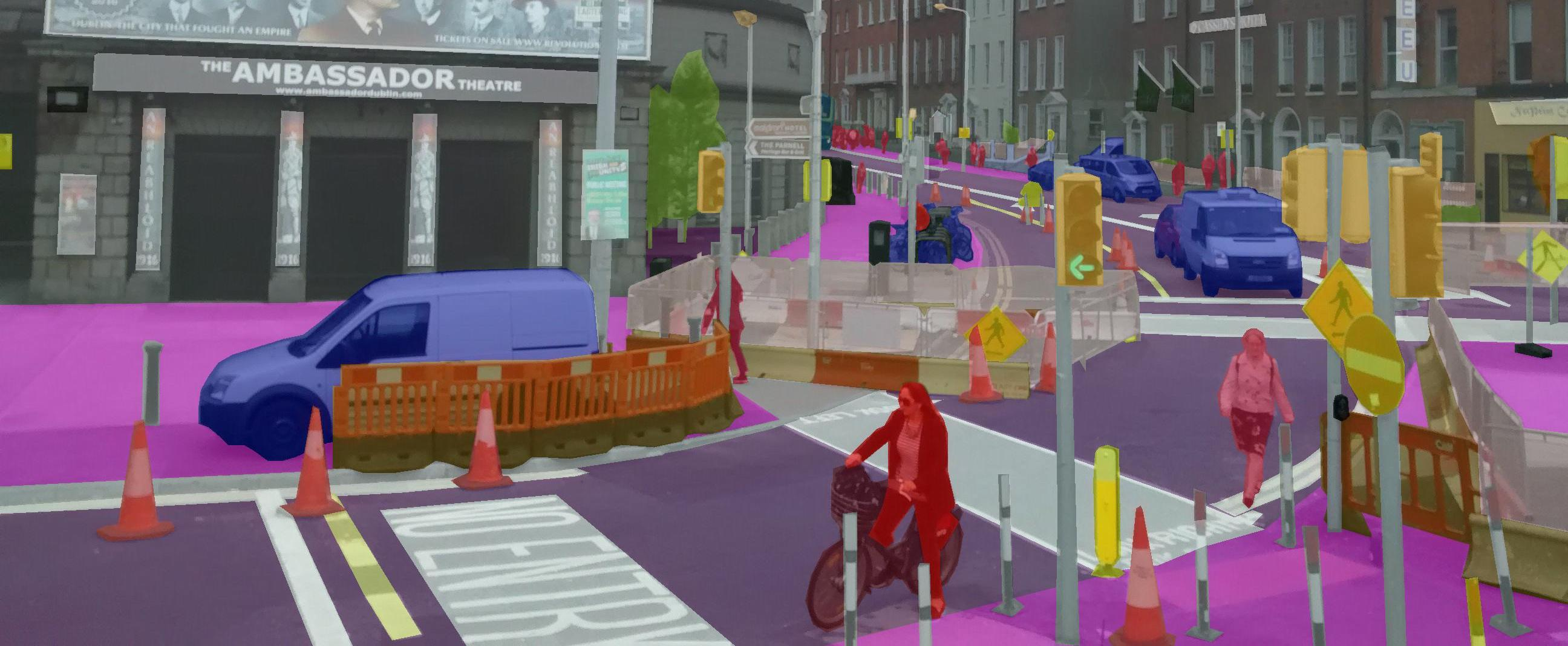Jan 29, 2018

## Introduction

Deep Convolution Neural Networks (DCNNs) have achieved remarkable success in various Computer Vision applications. Like others, the task of semantic segmentation is not an exception to this trend.

This piece provides an introduction to Semantic Segmentation with a hands-on TensorFlow implementation. We go over one of the most relevant papers on Semantic Segmentation of general objects - Deeplab_v3. You can clone the notebook for this post here.

## Semantic Segmentation 语义分割

Regular image classification DCNNs have similar structure. These models take images as input and output a single value representing the category of that image.

Usually, classification DCNNs have four main operations. Convolutionsactivation functionpooling, and fully-connected layers. Passing an image through a series of these operations outputs a feature vector containing the probabilities for each class label. Note that in this setup, we categorize an image as a whole. That is, we assign a single label to an entire image.Standard deep learning model for image recognition.

Image credits: Convolutional Neural Network MathWorks.

Different from image classification, in semantic segmentation we want to make decisions for every pixel in an image. So, for each pixel, the model needs to classify it as one of the pre-determined classes. Put another way, semantic segmentation means understanding images at a pixel level.

Keep in mind that semantic segmentation doesn’t differentiate between object instances. Here, we try to assign an individual label to each pixel of a digital image. Thus, if we have two objects of the same class, they end up having the same category label. Instance Segmentation is the class of problems that differentiate instances of the same class.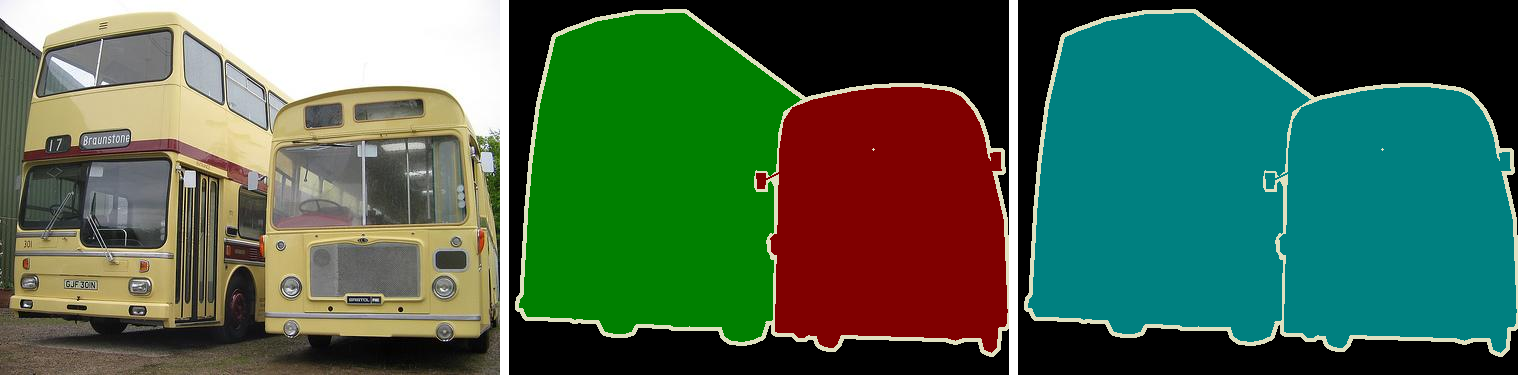Difference between Semantic Segmentation and Instance Segmentation. (middle) Although they are the same object (bus) they are classified as different objects. (left) Same object, equal category.

Yet, regular DCNNs such as the AlexNet and VGG aren’t suitable for dense prediction tasks. First, these models contain many layers designed to reduce the spatial dimensions of the input features. As a consequence, these layers end up producing highly decimated feature vectors that lack sharp details. Second, fully-connected layers have fixed sizes and loose spatial information during computation.

As an example, instead of having pooling and fully-connected layers, imagine passing an image through a series of convolutions. We can set each convolution to have stride of 1 and “SAME” padding. Doing this, each convolution preserves the spatial dimensions of its input. We can stack a bunch of these convolutions and have a segmentation model.Fully-Convolution neural network for dense prediction task. Note the non-existence of pooling and fully-connected layers.

This model could output a probability tensor with shape [W,H,C], where W and H represent the Width and Height. And C the number of class labels. Applying the argmax function (on the third axis) gives us a tensor shape of [W,H,1]. After, we compute the cross-entropy loss between each pixel of the ground-truth images and our predictions. In the end, we average that value and train the network using back prop.

There is one problem with this approach though. As we mentioned, using convolutions with stride 1 and “SAME” padding preserves the input dimensions. However, doing that would make the model super expensive in both ways. Memory consumption and computation complexity.

To ease that problem, segmentation networks usually have three main components. Convolutions, downsampling, and upsampling layers.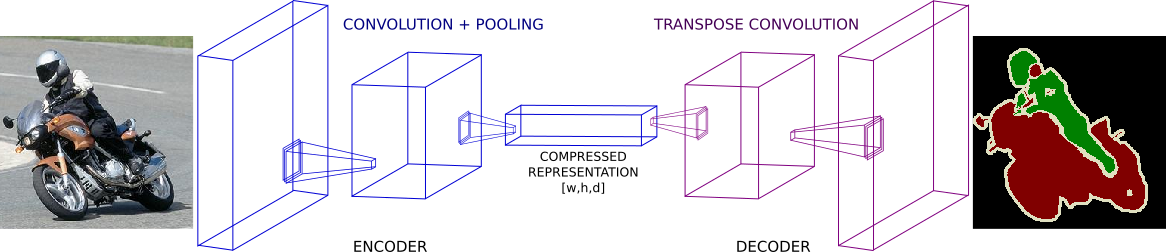Encoder-decoder architecture for Image Semantic Segmentation.

There are two common ways to do downsampling in neural nets. By using convolution striding or regular pooling operations. In general, downsampling has one goal. To reduce the spatial dimensions of given feature maps. For that reason, downsampling allows us to perform deeper convolutions without much memory concerns. Yet, they do it in detriment of losing some features in the process.

Also, note that the first part of this architecture looks a lot like usual classification DCNNs. With one exception, they do not put in place fully-connected layers.

After the first part, we have a feature vector with shape [w,h,d] where w, h and d are the width, height and depth of the feature tensor. Note that the spatial dimensions of this compressed vector are smaller (yet denser) than the original input.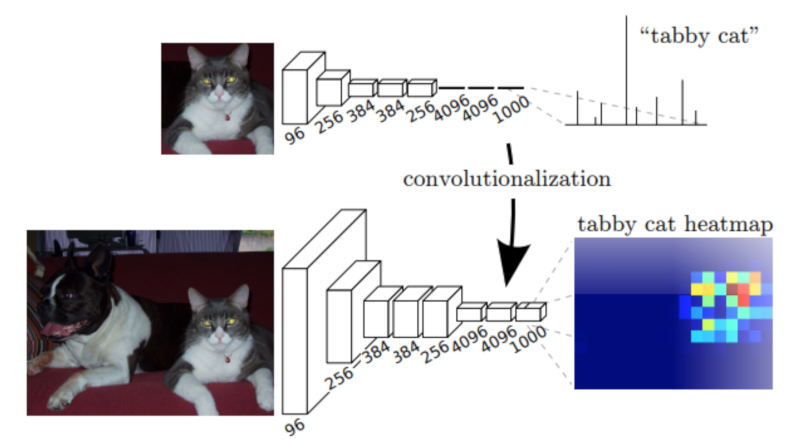(Top) VGG-16 network on its original form. Note the 3 fully-connected layers on top of the convolution stack. (Down) VGG-16 model when substituting its fully-connected layers to 1x1 convolutions. This change allows the network to output a coarse heat-map.

（顶部）VGG-16网络的原始形式。 注意卷积堆栈顶部的3个完全连接的层。 （下）VGG-16模型在将其全连接层替换为1x1卷积时。 此更改允许网络输出粗略的热图

Image credits: Fully Convolutional Networks for Semantic Segmentation.

At this point, regular classification DCNNs would output a dense (non-spatial) vector containing probabilities for each class label. Instead, we feed this compressed feature vector to a series of upsampling layers. These layers work on reconstructing the output of the first part of the network. The goal is to increase the spatial resolution so the output vector has the same dimensions as the input.

Usually, upsampling layers are based on strided transpose convolutionsThese functions go from deep and narrow layers to wider and shallower ones. Here, we use transpose convolutions to increase feature vectors dimension to the desired value.

In most papers, these two components of a segmentation network are called: encoder and decoder. In short, the first, “encodes” its information into a compressed vector used to represent its input. The second (the decoder) works on reconstructing this signal to the desired outcome.

There are many network implementations based on encoder-decoder architectures. FCNs, SegNetand UNet are some of the most popular ones. As a result, we have seen many successful segmentation models in a variety of fields.

## Model Architecture 模型架构

Different from most encoder-decoder designs, Deeplab offers a different approach to semantic segmentation. It presents an architecture for controlling signal decimation and learning multi-scale contextual features.Image credits: Rethinking Atrous Convolution for Semantic Image Segmentation.

Deeplab uses an ImageNet pre-trained ResNet as its main feature extractor network. However, it proposes a new Residual block for multi-scale feature learning. Instead of regular convolutions, the last ResNet block uses atrous convolutions. Also, each convolution (within this new block) uses different dilation rates to capture multi-scale context.

Deeplab使用ImageNet预训练的ResNet作为其主要特征提取器网络。 但是，它提出了一种用于多尺度特征学习的新残差块。 最后一个ResNet块不使用常规卷积，而是使用有害卷积。 此外，每个卷积（在此新块内）使用不同的扩张率来捕获多尺度上下文。

Additionally, on top of this new block, it uses Atrous Spatial Pyramid Pooling (ASPP). ASPP uses dilated convolutions with different rates as an attempt of classifying regions of an arbitrary scale.

To understand the deeplab architecture, we need to focus on three components. (i) The ResNet architecture, (ii) atrous convolutions and (iii) Atrous Spatial Pyramid Pooling (ASPP). Let’s go over each one of them.

### ResNets

ResNet is a very popular DCNN that won the ILSVRC 2015 classification task. One of the main contributions of ResNets was to provide a framework to ease the training of deeper models.

ResNet是一个非常受欢迎的DCNN，赢得了ILSVRC 2015分类任务。 ResNets的主要贡献之一是提供一个框架来简化深层模型的培训。

In its original form, ResNets contain 4 computational blocks. Each block contains a different number of Residual Units. These units perform a series of convolutions in a special way. Also, each block is intercalated with max-pooling operations to reduce spatial dimensions.

The original paper presents two types of Residual Units. The baseline and the bottleneck blocks.

The baseline unit contains two 3x3 convolutions with Batch Normalization(BN) and ReLU activations.ResNet building blocks. (left) baseline; (right) bottleneck unit

Adapted from: Deep Residual Learning for Image Recognition.

The second, the bottleneck unit, consists of three stacked operations. A series of 1x13x3 and 1x1convolutions substitute the previous design. The two 1x1 operations are designed for reducing and restoring dimensions. This leaves the 3x3 convolution, in the middle, to operate on a less dense feature vector. Also, BN is applied after each convolution and before ReLU non-linearity.

To help to understand, let’s denote these group of operations as a function F of its input x.

After the non-linear transformations in F(x), the unit combines the result of F(x) with the original input x. This combination is done by adding the two functions. Merging the original input x with the non-linear function F(x) offers some advantages. It allows earlier layers to access the gradient signal from later layers. In other words, skipping the operations on F(x) allows earlier layers to have access to a stronger gradient signal. As a result, this type of connectivity has been shown to ease the training of deeper networks.

Non-bottleneck units also show gain in accuracy as we increase model capacity. Yet, bottleneck residual units have some practical advantages. First, it performs more computations having almost the same number of parameters. Second, they also perform in a similar computational complexity as its counterpart.

In practice, bottleneck units are more suitable for training deeper models because of less training time and computational resources need.

For our implementation, we use the full pre-activation Residual Unit. The only difference, from the standard bottleneck unit, lies in the order in which BN and ReLU activations are placed. For the full pre-activation, BN and ReLU (in this order) occur before convolutions.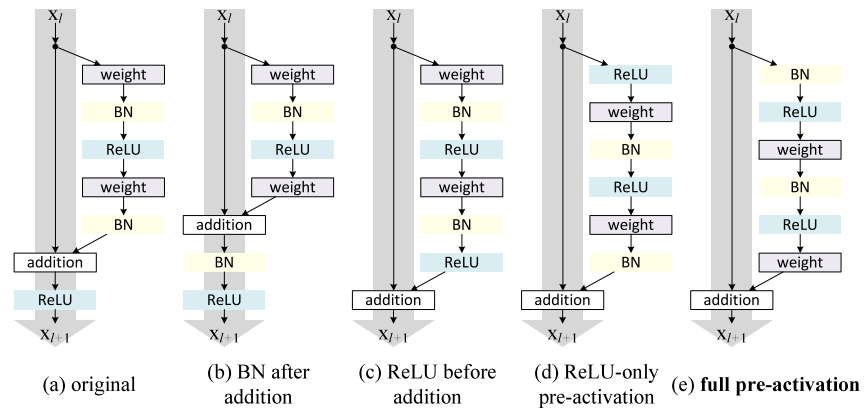Different ResNet building block architectures. (Left-most) the original ResNet block. (Right-most) the improved full pre-activation version.

Image credits: Identity Mappings in Deep Residual Networks.

As shown in Identity Mappings in Deep Residual Networks, the full pre-activation unit performs better than other variants.

Note that the only difference between these designs is the order of BN and RELu in the convolution stack.

### Atrous Convolutions

Atrous (or dilated) convolutions are regular convolutions with a factor that allows us to expand the filter’s field of view.

Atrous（或扩张）卷积是常规卷积，其中一个因子允许我们扩展滤波器的视野。

Consider a 3x3 convolution filter for instance. When the dilation rate is equal to 1, it behaves like a standard convolution. But, if we set the dilation factor to 2, it has the effect of enlarging the convolution kernel.

In theory, it works like that. First, it expands (dilates) the convolution filter according to the dilation rate. Second, it fills the empty spaces with zeros - creating a sparse like filter. Finally, it performs regular convolution using the dilated filter.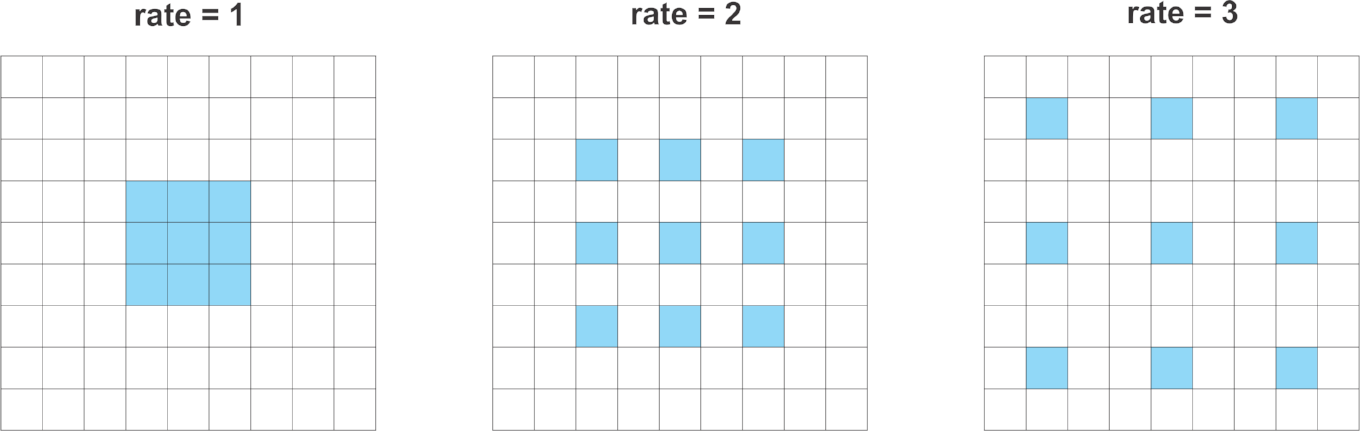Atrous convolutions with various rates.  各种费率的萎缩卷积。

As a consequence, a convolution with a dilated 2, 3x3 filter would make it able to cover an area equivalent to a 5x5. Yet, because it acts like a sparse filter, only the original 3x3 cells will do computation and produce results. I said “act” because most frameworks don’t implement atrous convolutions using sparse filters - because of memory concerns.

In a similar way, setting the atrous factor to 3 allows a regular 3x3 convolution to get signals from a 7x7 corresponding area.

This effect allows us to control the resolution at which we compute feature responses. Also, atrous convolution adds larger context without increasing the number of parameters or the amount of computation.

Deeplab also shows that the dilation rate must be tuned according to the size of the feature maps. They studied the consequences of using large dilation rates over small feature maps.

Deeplab还表明，必须根据要素图的大小调整扩张率。 他们研究了在小特征图上使用大的扩张率的后果。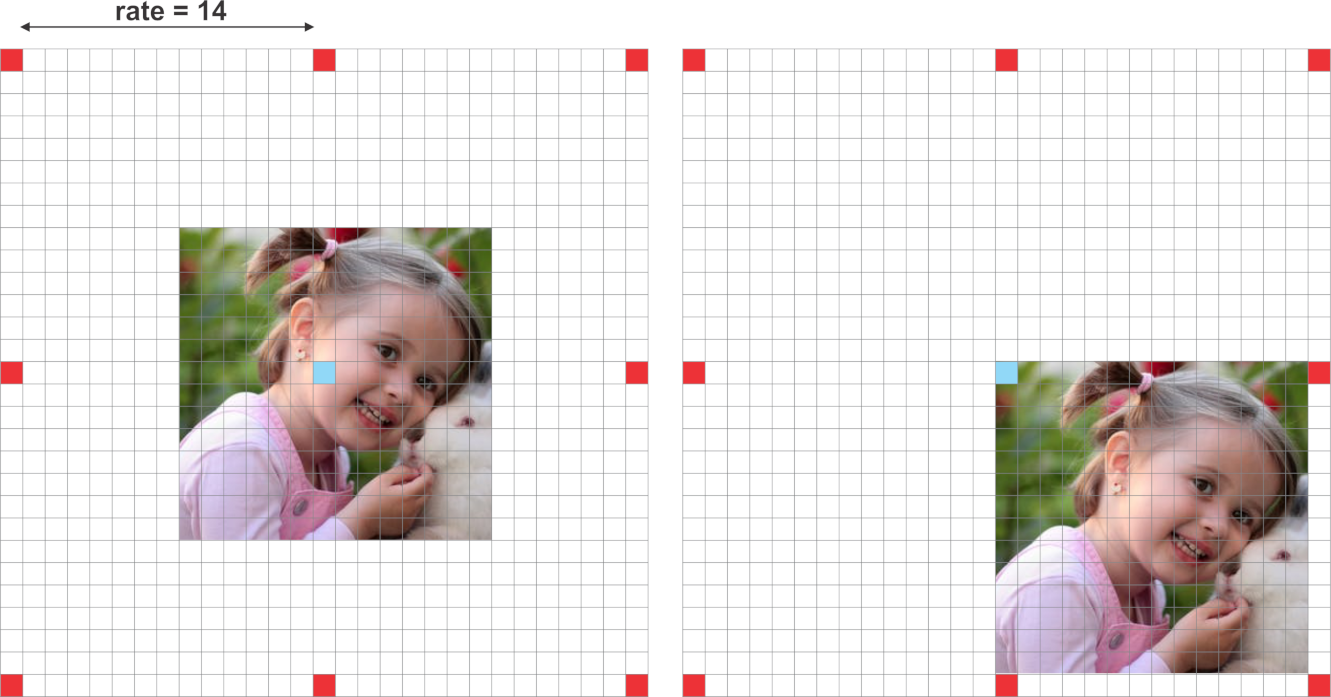Side effects of setting larger dilation rates for smaller feature maps. For a 14x14 input image, a *3x3* filter with dilation rate of 15 makes the atrous convolution behaves like a regular 1x1 convolution.

When the dilation rate is very close to the feature map’s size, a regular 3x3 atrous filter acts as a standard 1x1 convolution.

Put in another way, the efficiency of atrous convolutions depends on a good choice of the dilation rate. Because of that, it is important to know the concept of output stride in neural networks.

Output stride explains the ratio of the input image size to the output feature map size. It defines how much signal decimation the input vector suffers as it passes the network.

For an output stride of 16, an image size of 224x224x3 outputs a feature vector with 16 times smaller dimensions. That is 14x14.

Besides, Deeplab also debates the effects of different output strides on segmentation models. It argues that excessive signal decimation is harmful for dense prediction tasks. In short, models with smaller output stride - less signal decimation - tends to output finer segmentation results. Yet, training models with smaller output stride demand more training time.

Deeplab报告了两种输出步幅配置的实验，8和16.正如预期的那样，输出stride = 8能够产生稍好的结果。这里我们选择输出stride = 16出于实际原因。

Also, because the atrous block doesn’t implement downsampling, ASPP also runs on the same feature response size. As a result, it allows learning features from multi-scale context using relative large dilation rates.

The new Atrous Residual Block contains three residual units. In total, the 3 units have three 3x3convolutions. Motivated by multigrid methods, Deeplab proposes different dilation rates for each convolution. In summary, multigrid defines the dilation rates for each of the three convolutions.

In practice:

For the new block4, when output stride = 16 and Multi Grid = (1, 2, 4), the three convolutions have rates = 2 · (1, 2, 4) = (2, 4, 8) respectively.

### Atrous Spatial Pyramid Pooling

For ASPP, the idea is to provide the model with multi-scale information. To do that, ASPP adds a series atrous convolutions with different dilation rates. These rates are designed to capture long-range context. Also, to add global context information, ASPP incorporates image-level features via Global Average Pooling (GAP).

This version of ASPP contains 4 parallel operations. These are a 1x1 convolution and three 3x3convolutions with dilation rates =(6,12,18). As we mentioned, at this point, the feature maps’ nominal stride is equal to 16.

Based on the original implementation, we use crop sizes of 513x513 for both: training and testing. Thus, using an output stride 16 means that ASPP receives feature vectors of size 32x32.

@slim.add_arg_scope
def atrous_spatial_pyramid_pooling(net, scope, depth=256):
"""
ASPP consists of (a) one 1×1 convolution and three 3×3 convolutions with rates = (6, 12, 18) when output stride = 16
(all with 256 filters and batch normalization), and (b) the image-level features as described in https://arxiv.org/abs/1706.05587
:param net: tensor of shape [BATCH_SIZE, WIDTH, HEIGHT, DEPTH]
:param scope: scope name of the aspp layer
:return: network layer with aspp applyed to it.
"""

with tf.variable_scope(scope):
feature_map_size = tf.shape(net)

# apply global average pooling
image_level_features = tf.reduce_mean(net, [1, 2], name='image_level_global_pool', keep_dims=True)
image_level_features = slim.conv2d(image_level_features, depth, [1, 1], scope="image_level_conv_1x1", activation_fn=None)
image_level_features = tf.image.resize_bilinear(image_level_features, (feature_map_size, feature_map_size))

at_pool1x1 = slim.conv2d(net, depth, [1, 1], scope="conv_1x1_0", activation_fn=None)

at_pool3x3_1 = slim.conv2d(net, depth, [3, 3], scope="conv_3x3_1", rate=6, activation_fn=None)

at_pool3x3_2 = slim.conv2d(net, depth, [3, 3], scope="conv_3x3_2", rate=12, activation_fn=None)

at_pool3x3_3 = slim.conv2d(net, depth, [3, 3], scope="conv_3x3_3", rate=18, activation_fn=None)

net = tf.concat((image_level_features, at_pool1x1, at_pool3x3_1, at_pool3x3_2, at_pool3x3_3), axis=3,
name="concat")
net = slim.conv2d(net, depth, [1, 1], scope="conv_1x1_output", activation_fn=None)
return net

In the end, the features, from all the branches, are combined into a single vector via concatenation. This output is then convoluted with another 1x1 kernel - using BN and 256 filters.

After ASPP, we feed the result to another 1x1 convolution - to produce the final segmentation logits.

## Implementation Details

Using the ResNet-50 as feature extractor, this implementation of Deeplab_v3 employs the following network configuration:

• output stride = 16     输出stride = 16
• Fixed multi-grid atrous convolution rates of (1,2,4) to the new Atrous Residual block (block 4).
• 修复了（1,2,4）新的Atrous残余块的多网格迂回卷积率（方框4）。
• ASPP with rates (6,12,18) after the last Atrous Residual block.
• ASPP在最后一次Atrous Residual块之后的费率（6,12,18）。

Setting output stride to 16 gives us the advantage of substantially faster training. Comparing to output stride of 8, stride of 16 makes the Atrous Residual block deals with 4 times smaller feature maps than its counterpart.

The multi-grid dilation rates are applied to the 3 convolutions inside the Atrous Residual block.

Finally, each of the three parallel 3x3 convolutions in ASPP gets a different dilation rate - (6,12,18).

Before computing the cross-entropy error, we resize the logits to the input’s size. As argued in the paper, it’s better to resize the logits than the ground-truth labels to keep resolution details.

Based on the original training procedures, we scale each image using a random factor from 0.5 to 2. Also, we apply random left-right flipping to the scaled images.

Finally, we crop patches of size 513x513 for both training and testing.

def deeplab_v3(inputs, args, is_training, reuse):

# mean subtraction normalization
inputs = inputs - [_R_MEAN, _G_MEAN, _B_MEAN]

# inputs has shape [batch, 513, 513, 3]
with slim.arg_scope(resnet_utils.resnet_arg_scope(args.l2_regularizer, is_training,
args.batch_norm_decay,
args.batch_norm_epsilon)):
resnet = getattr(resnet_v2, args.resnet_model) # get one of the resnet models: resnet_v2_50, resnet_v2_101 ...
_, end_points = resnet(inputs,
args.number_of_classes,
is_training=is_training,
global_pool=False,
spatial_squeeze=False,
output_stride=args.output_stride,
reuse=reuse)

with tf.variable_scope("DeepLab_v3", reuse=reuse):

# get block 4 feature outputs
net = end_points[args.resnet_model + '/block4']

net = atrous_spatial_pyramid_pooling(net, "ASPP_layer", depth=256, reuse=reuse)

net = slim.conv2d(net, args.number_of_classes, [1, 1], activation_fn=None,
normalizer_fn=None, scope='logits')

size = tf.shape(inputs)[1:3]
# resize the output logits to match the labels dimensions
#net = tf.image.resize_nearest_neighbor(net, size)
net = tf.image.resize_bilinear(net, size)
return net

To implement atrous convolutions with multi-grid in the block4 of the resnet, we just changed this piece in the resnet_utils.py file.

...
with tf.variable_scope('unit_%d' % (i + 1), values=[net]):
# If we have reached the target output_stride, then we need to employ
# atrous convolution with stride=1 and multiply the atrous rate by the
# current unit's stride for use in subsequent layers.
if output_stride is not None and current_stride == output_stride:
# Only uses atrous convolutions with multi-graid rates in the last (block4) block
if block.scope == "block4":
net = block.unit_fn(net, rate=rate * multi_grid[i], **dict(unit, stride=1))
else:
net = block.unit_fn(net, rate=rate, **dict(unit, stride=1))
rate *= unit.get('stride', 1)
...

## Training

To train the network, we decided to use the augmented Pascal VOC dataset provided by Semantic contours from inverse detectors. 为了训练网络，我们决定使用来自反向探测器的语义轮廓提供的增强Pascal VOC数据集。

The training data is composed of 8,252 images. 5,623 from the training set and 2,299 from the validation set. To test the model using the original VOC 2012 val dataset, I removed 558 images from the 2,299 validation set. These 558 samples were also present on the official VOC validation set. Also, I added 330 images from the VOC 2012 train set that weren’t present either among the 5,623 nor the 2,299 sets. Finally, 10% of the 8,252 images (~825 samples) are held for validation, leaving the rest for training.

Note that different from the original paper, this implementation is not pre-trained in the COCO dataset. Also, some of the techniques described in the paper for training and evaluation were not queried out.

## Results

The model was able to achieve decent results on the PASCAL VOC validation set.

• Pixel accuracy: ~91%
• Mean Accuracy: ~82%
• Mean Intersection over Union (mIoU): ~74%
• Frequency weighed Intersection over Union: ~86%.

Bellow, you can check out some of the results in a variety of images from the PASCAL VOC validation set.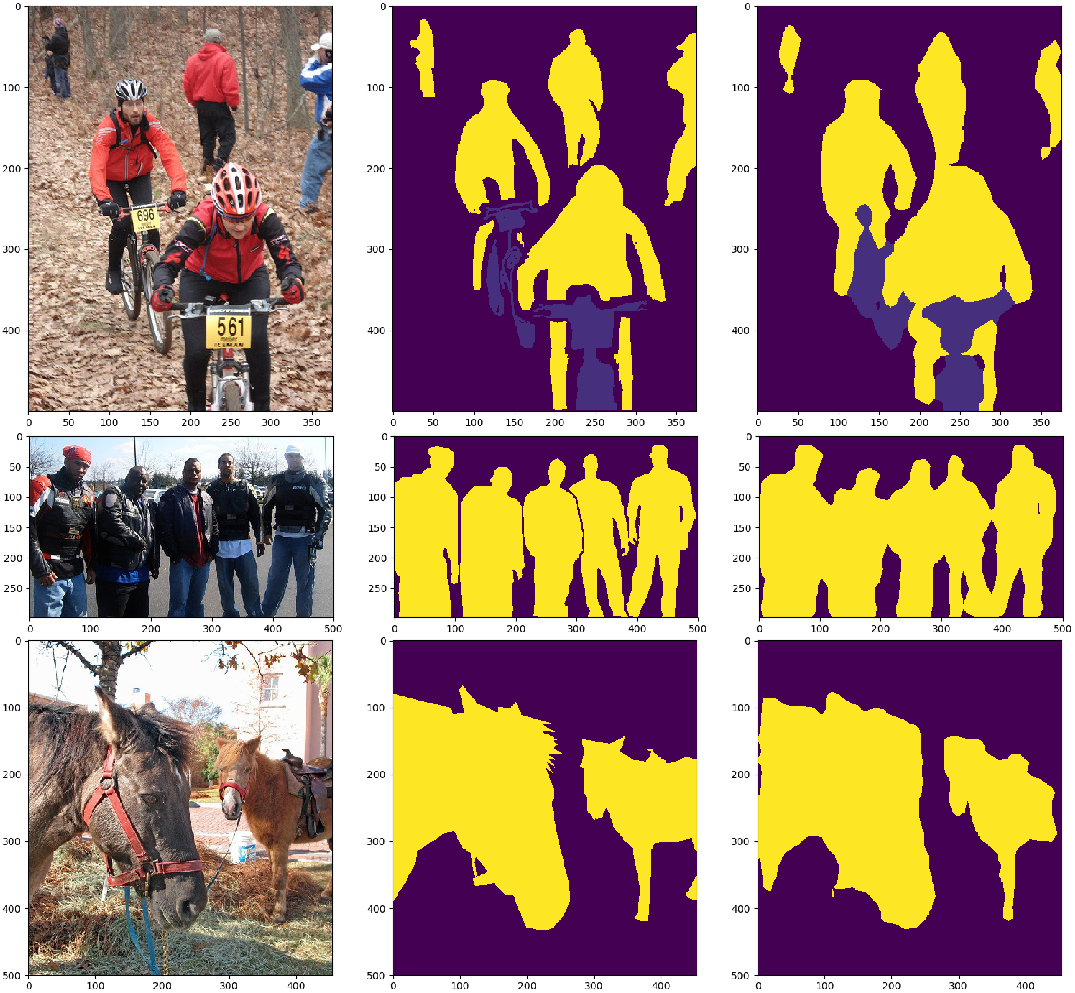## Concluding

The field of Semantic Segmentation is no doubt one of the hottest ones in Computer Vision. Deeplab presents an alternative to classic encoder-decoder architectures. It advocates the usage of atrous convolutions for feature learning in multi-range contexts. Feel free to clone the repo and tune the model to achieve closer results to the original implementation. The complete code is here.